# Concise Selina Solutions for Class 9 Maths Chapter 9- Triangles [Congruency in Triangles]

The Selina solutions for the questions given in chapter 9, Triangles, of the Class 9 Selina textbooks are available here. In this chapter students learn about the topic of Triangles, mainly focussing on Congruency in Triangles, in detail. Solving all the questions present in the Selina textbook will help the students in scoring full marks in the exam.

The Class 9 Selina solutions maths are very easy to understand. These solutions cover all the exercise questions included in the book and are in accordance to the syllabus prescribed by the ICSE or CISCE. Here, the PDF of the Class 9 Maths chapter 9 Selina solutions is available which can be downloaded as well as viewed online. Students can also avail these Selina solutions and download it for free to practice them offline as well.

## Download PDF of Selina Solutions for Class 9 Maths Chapter 9:-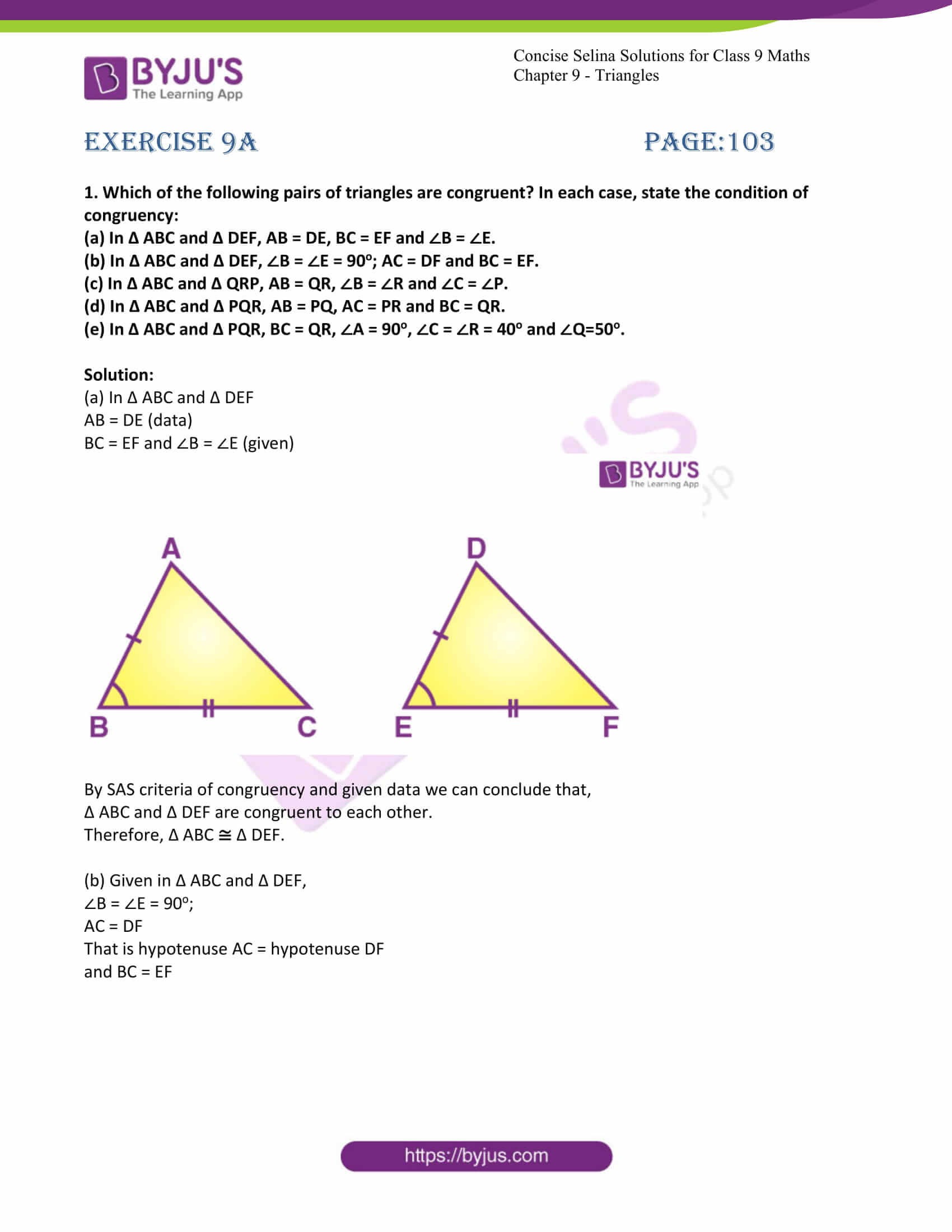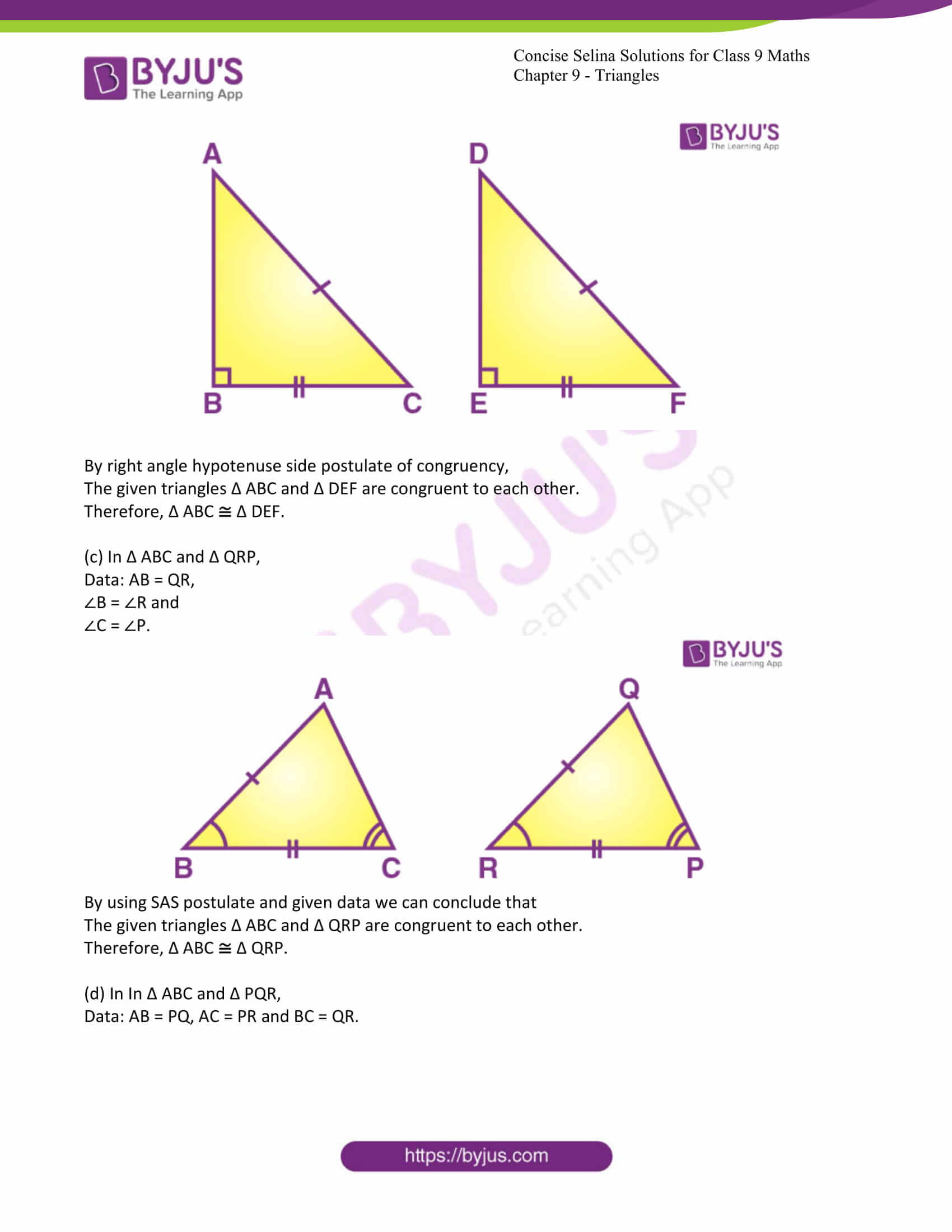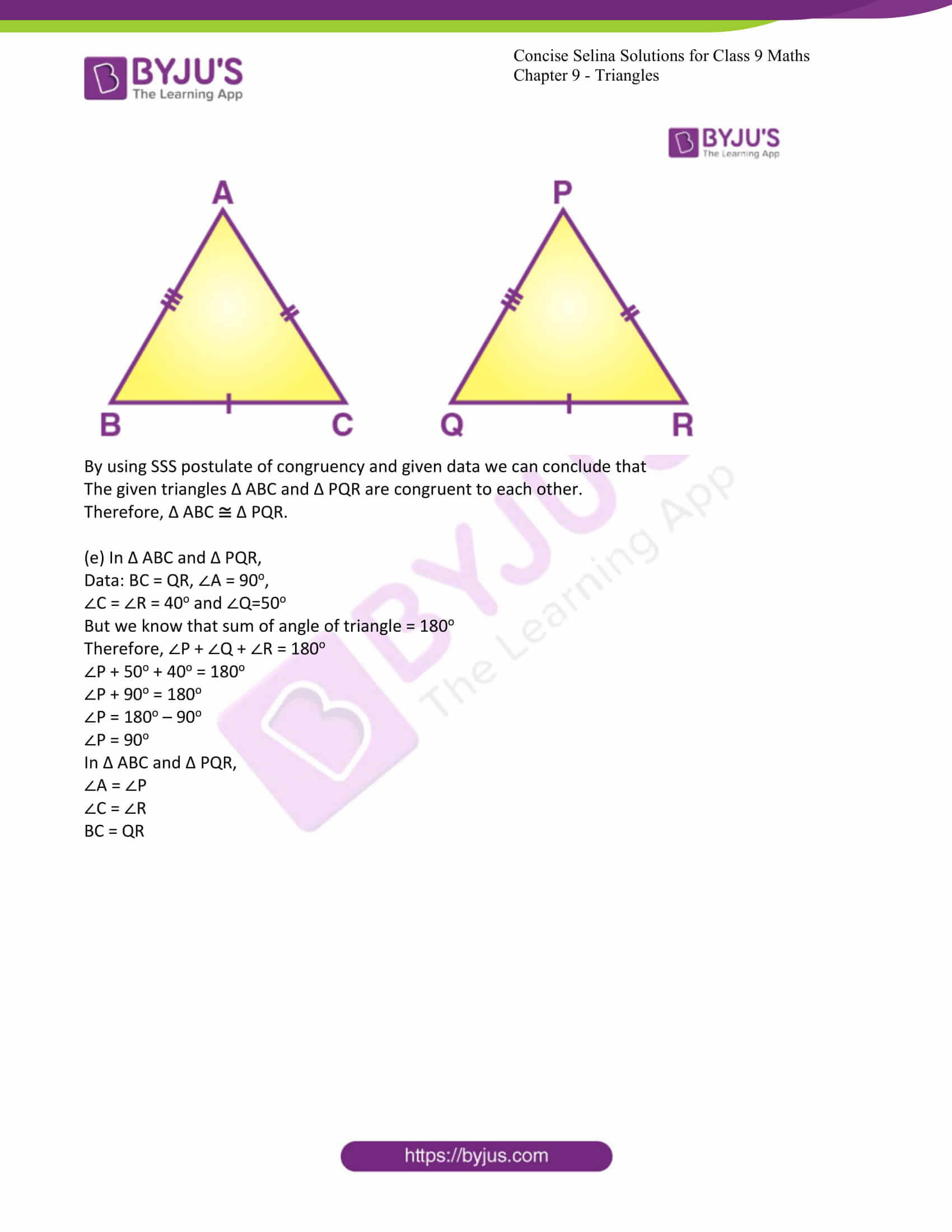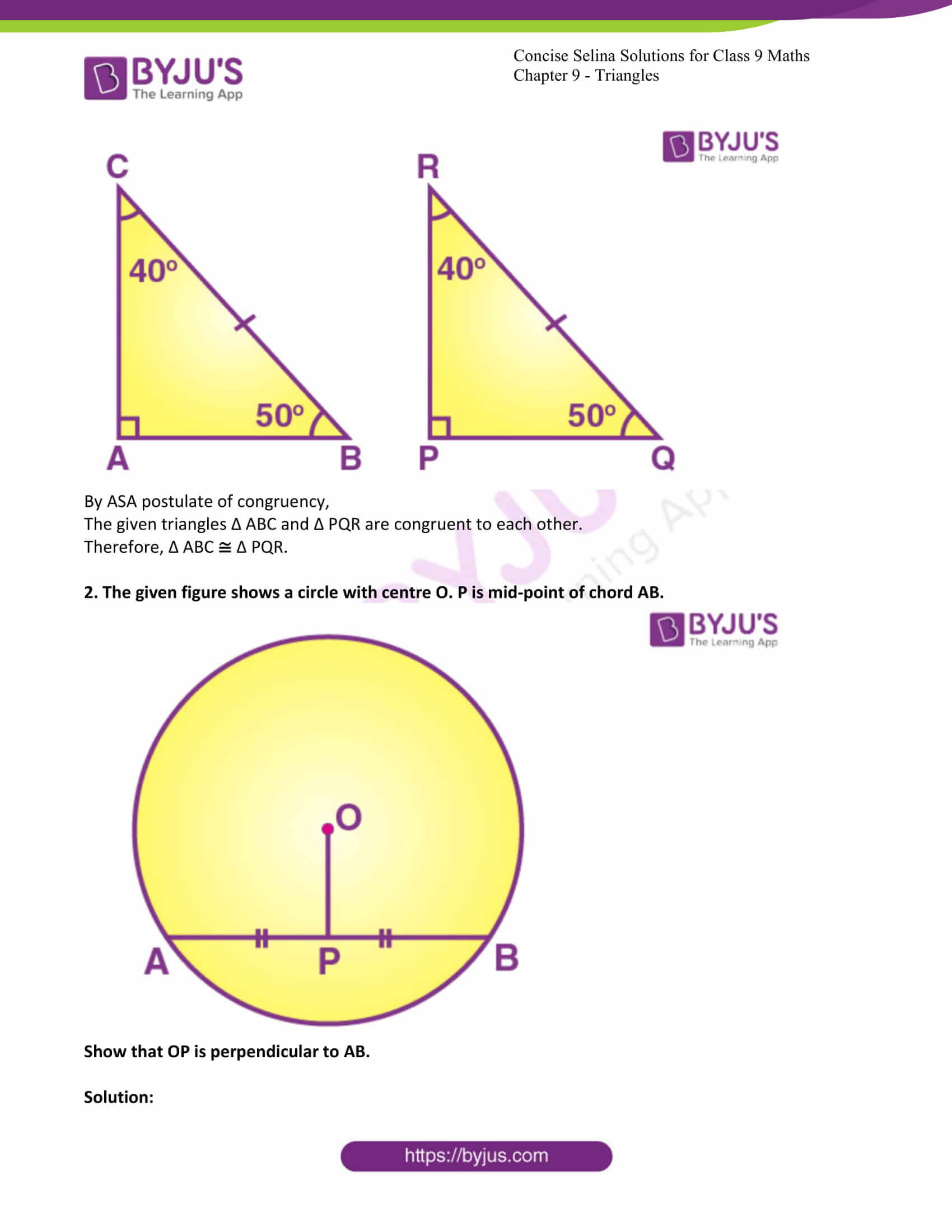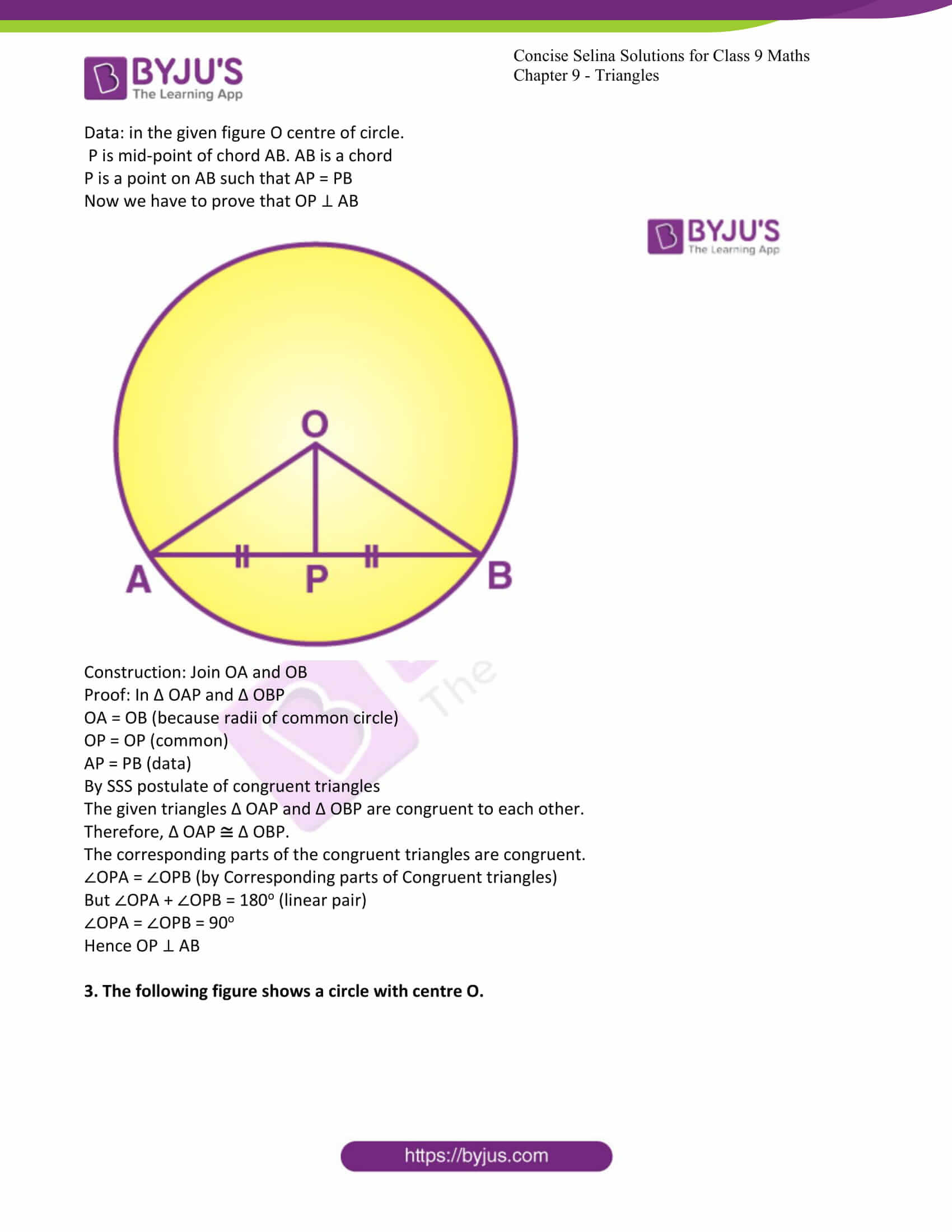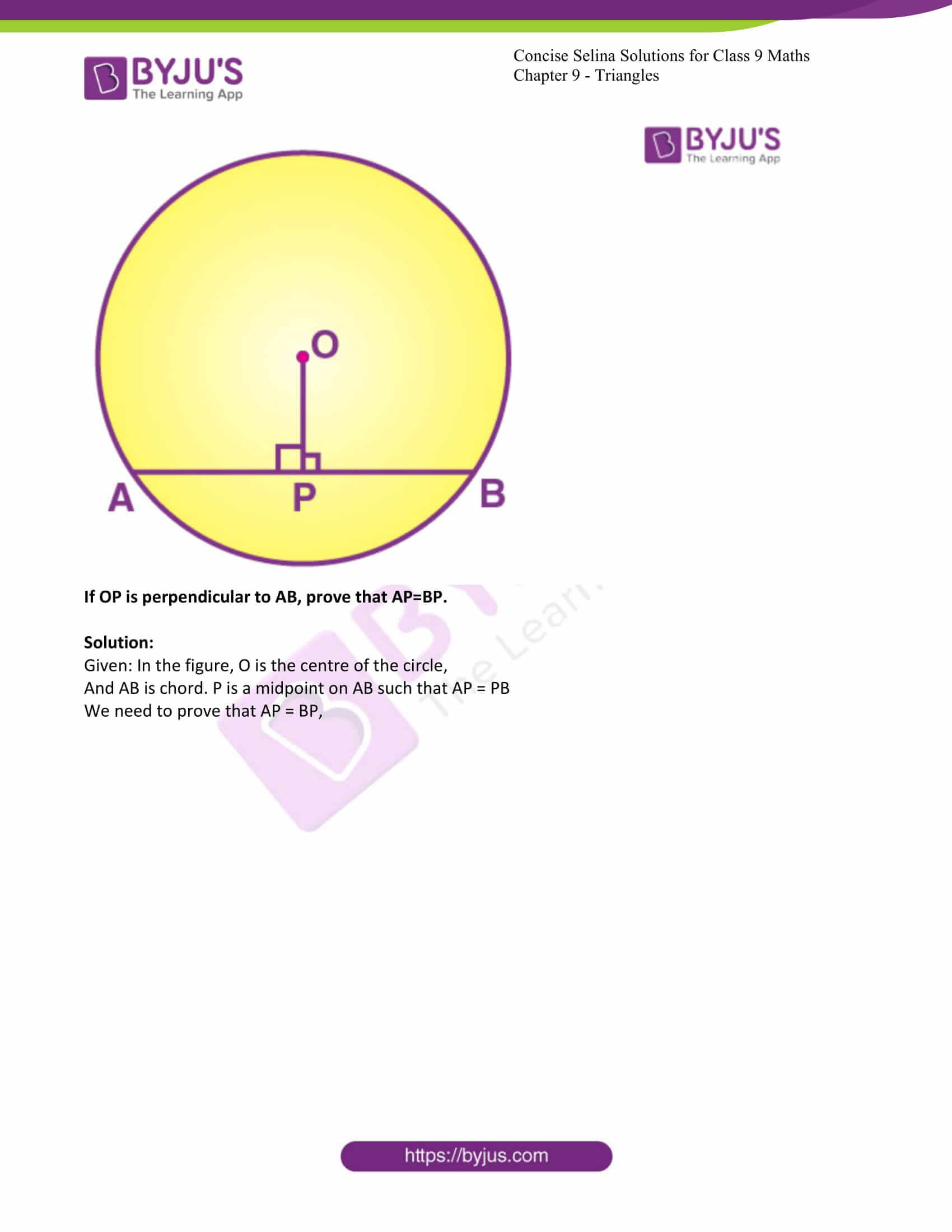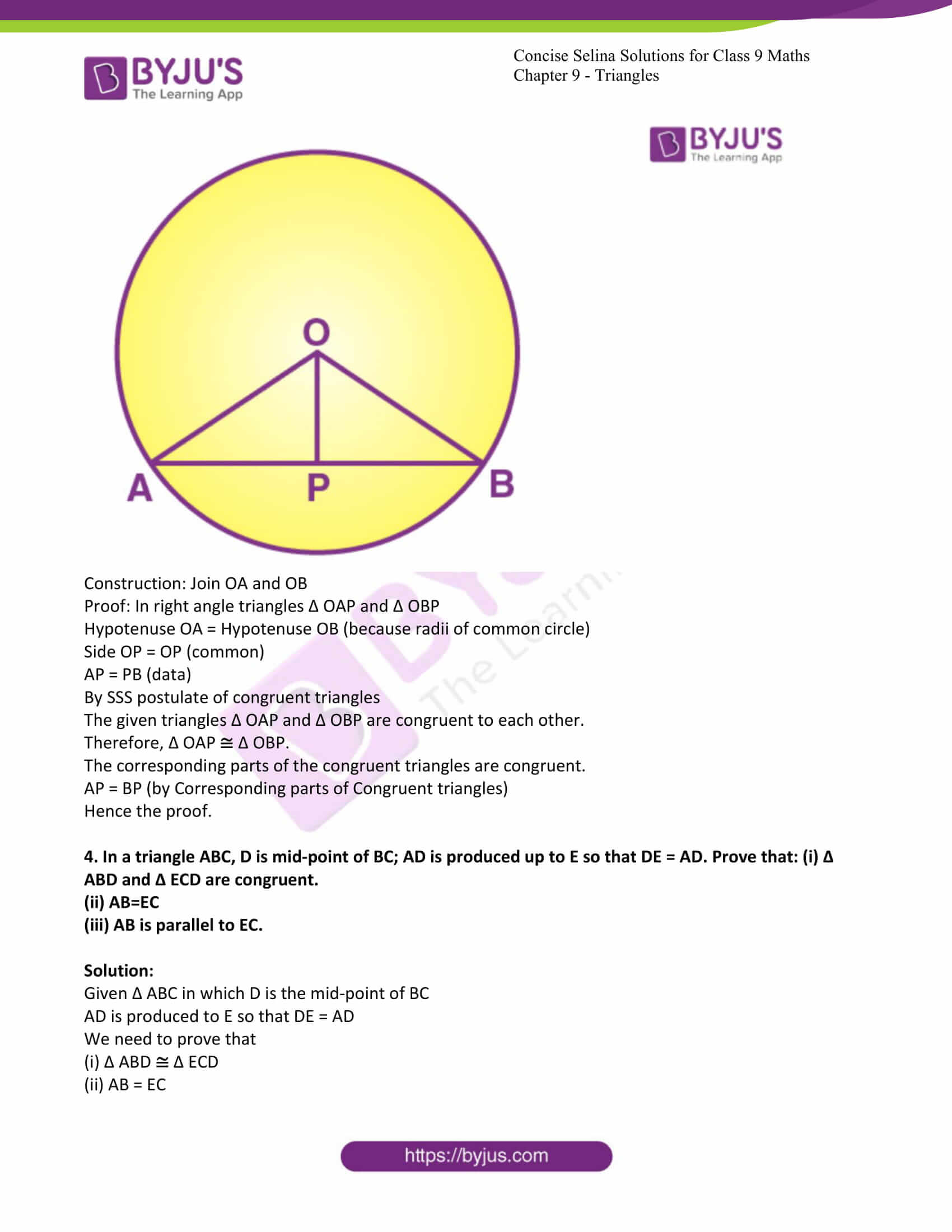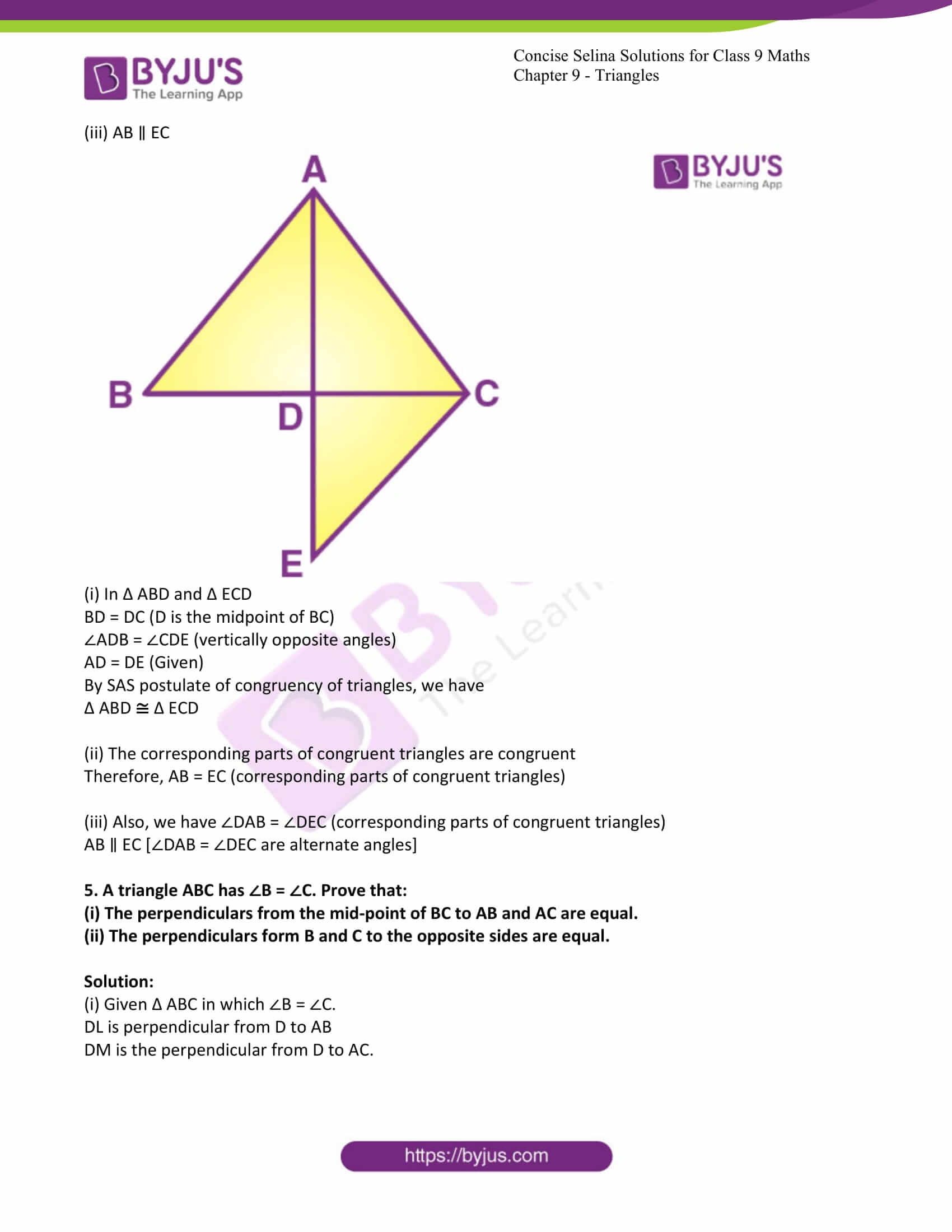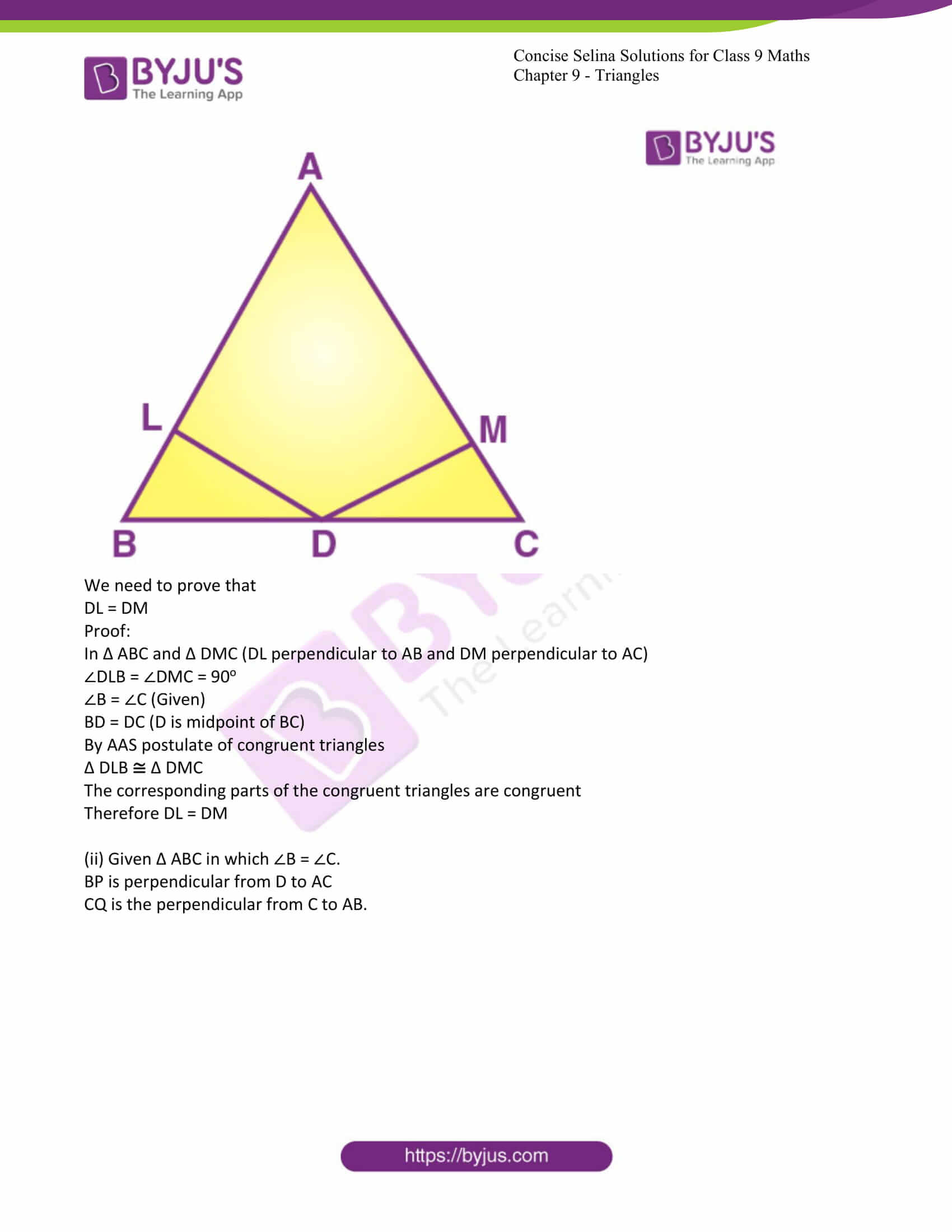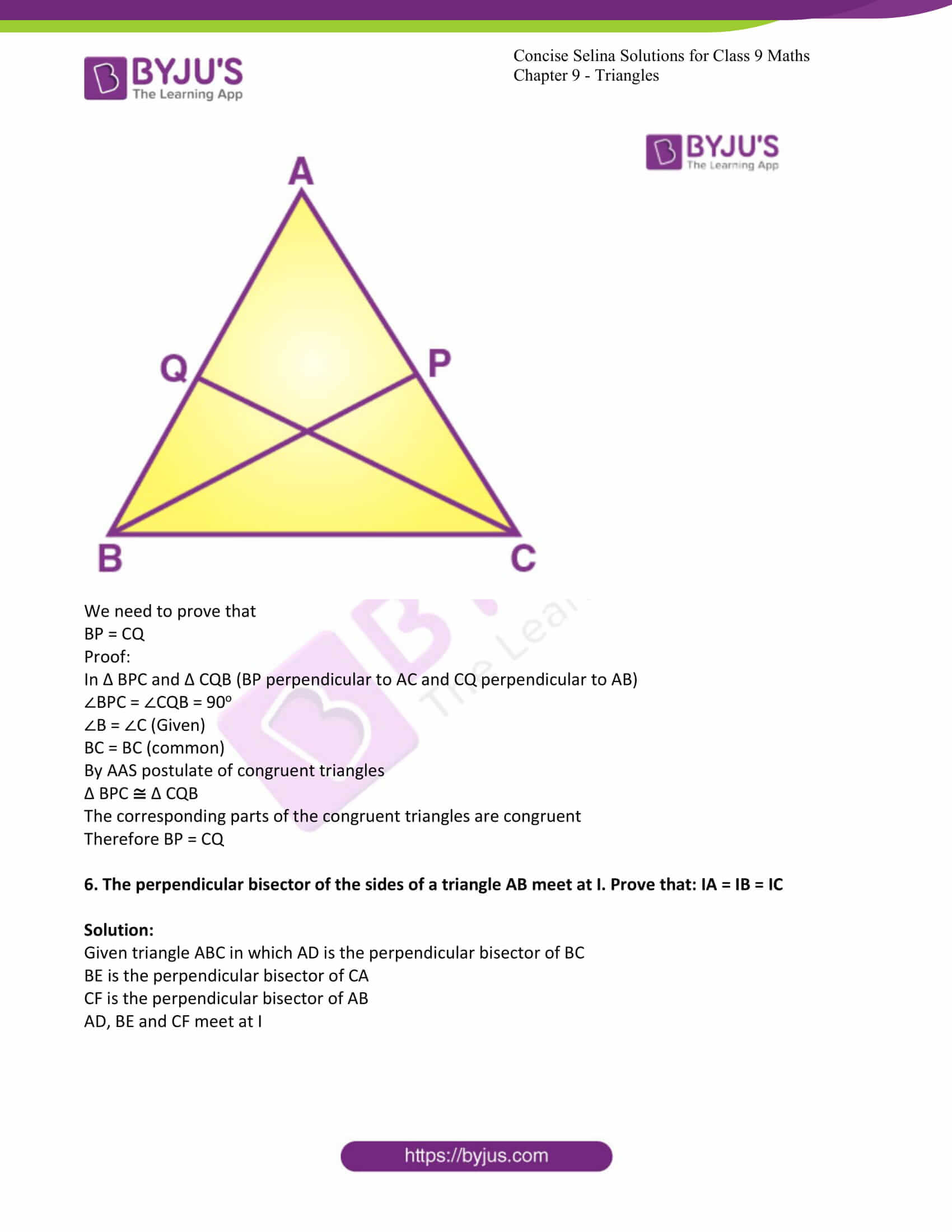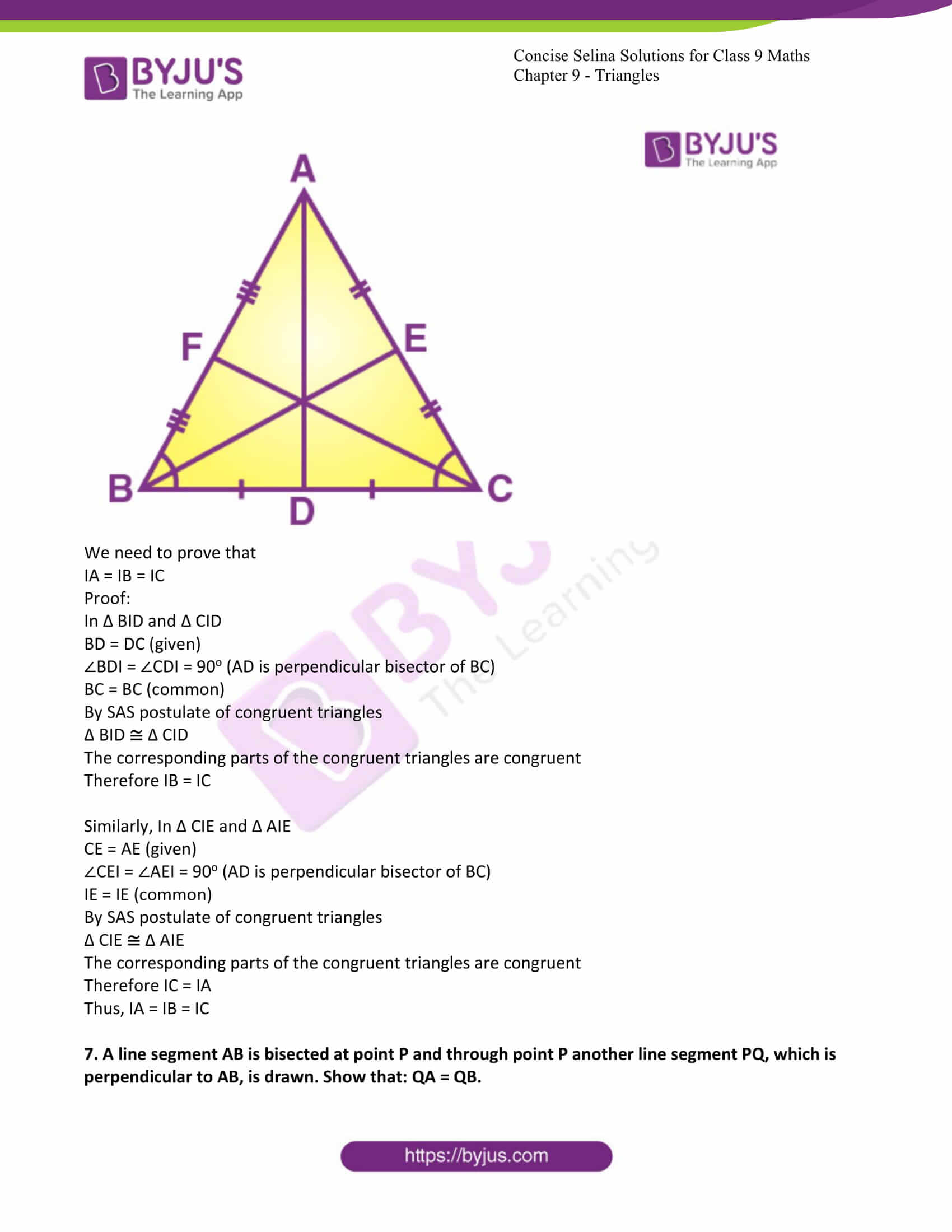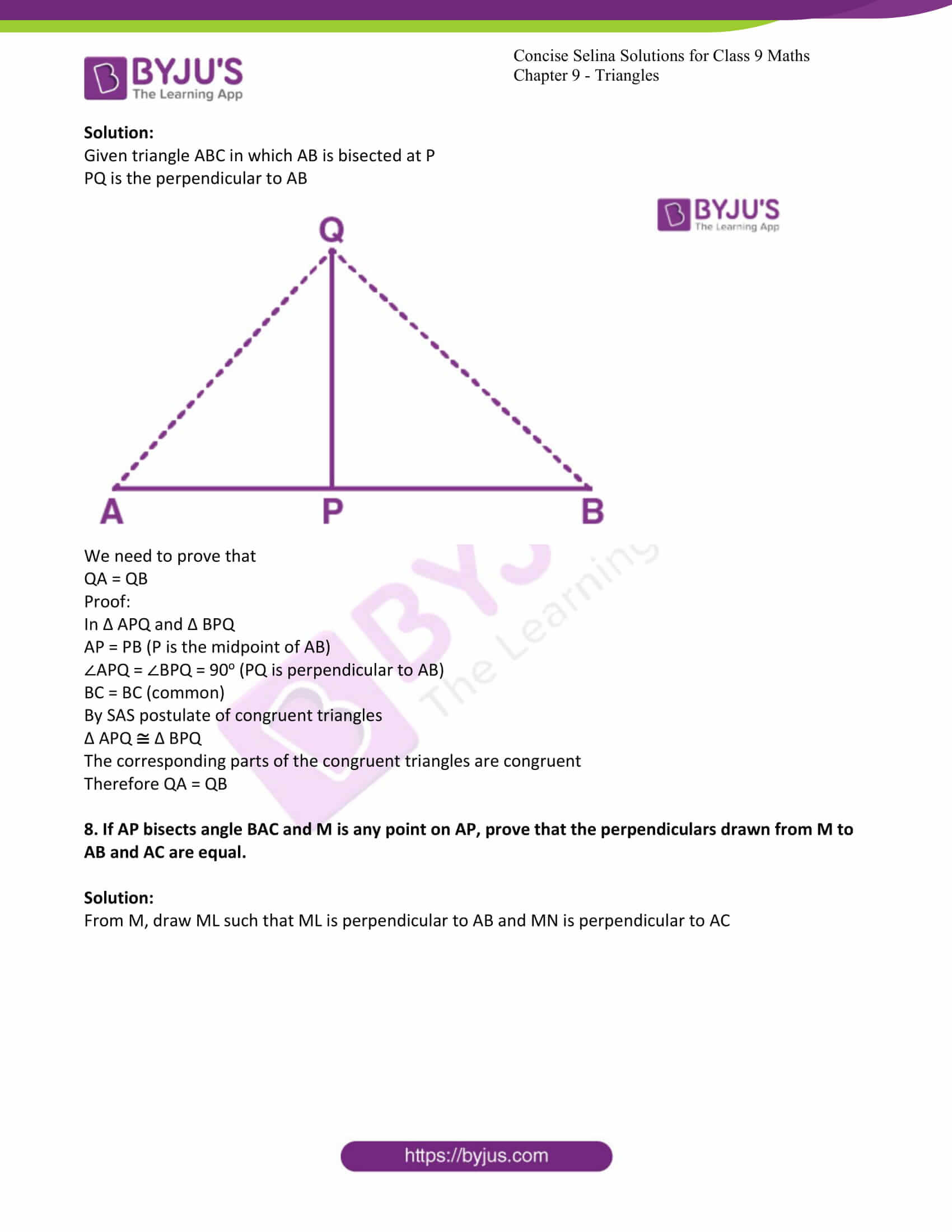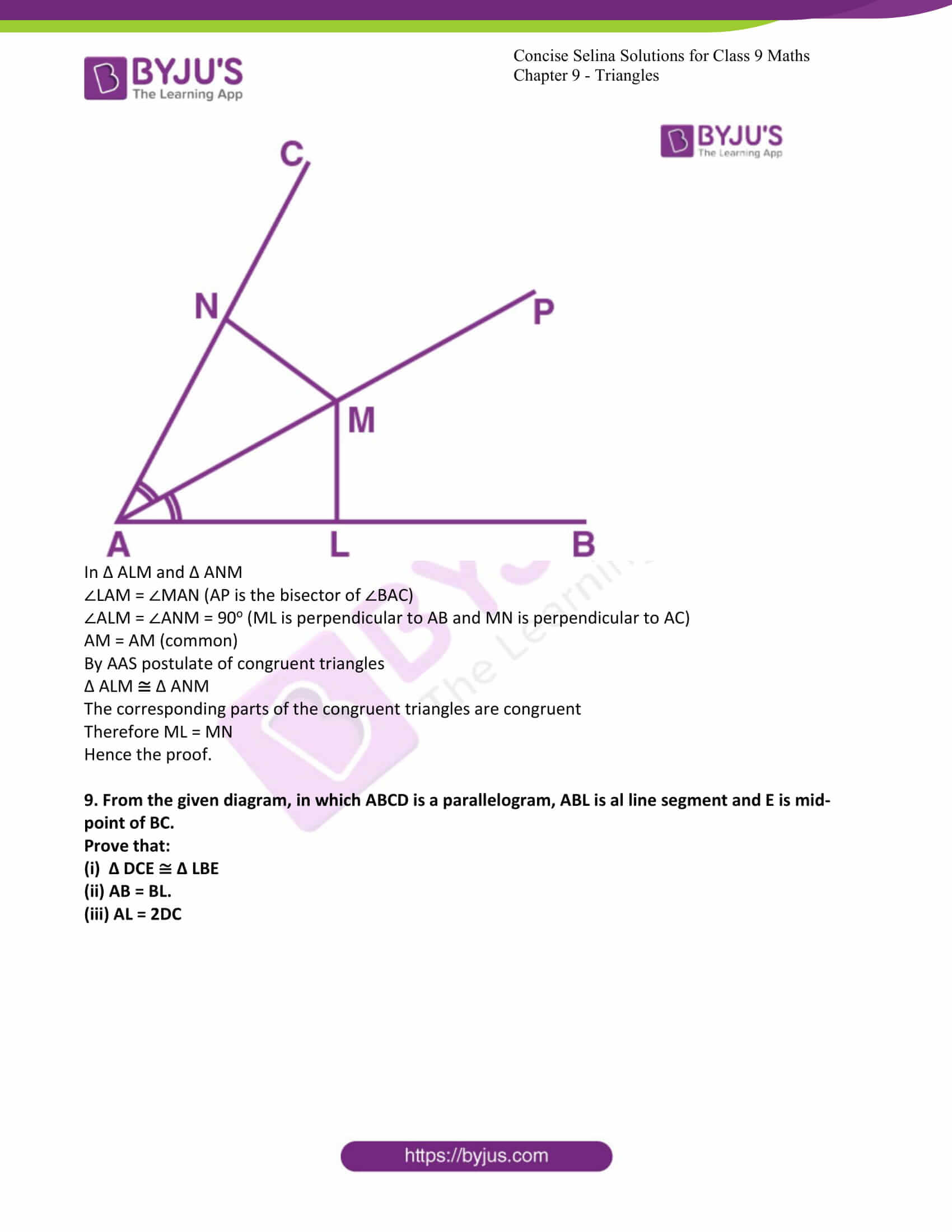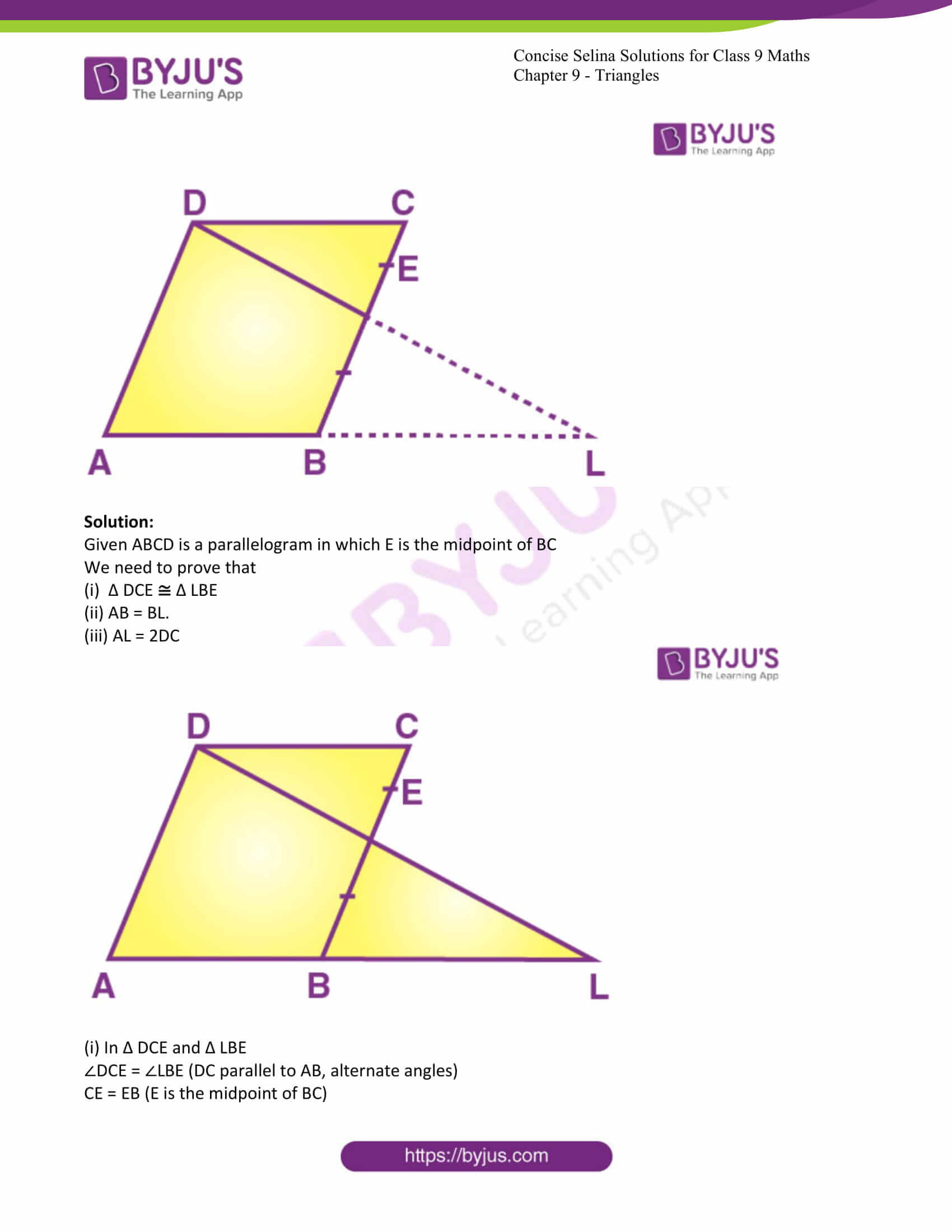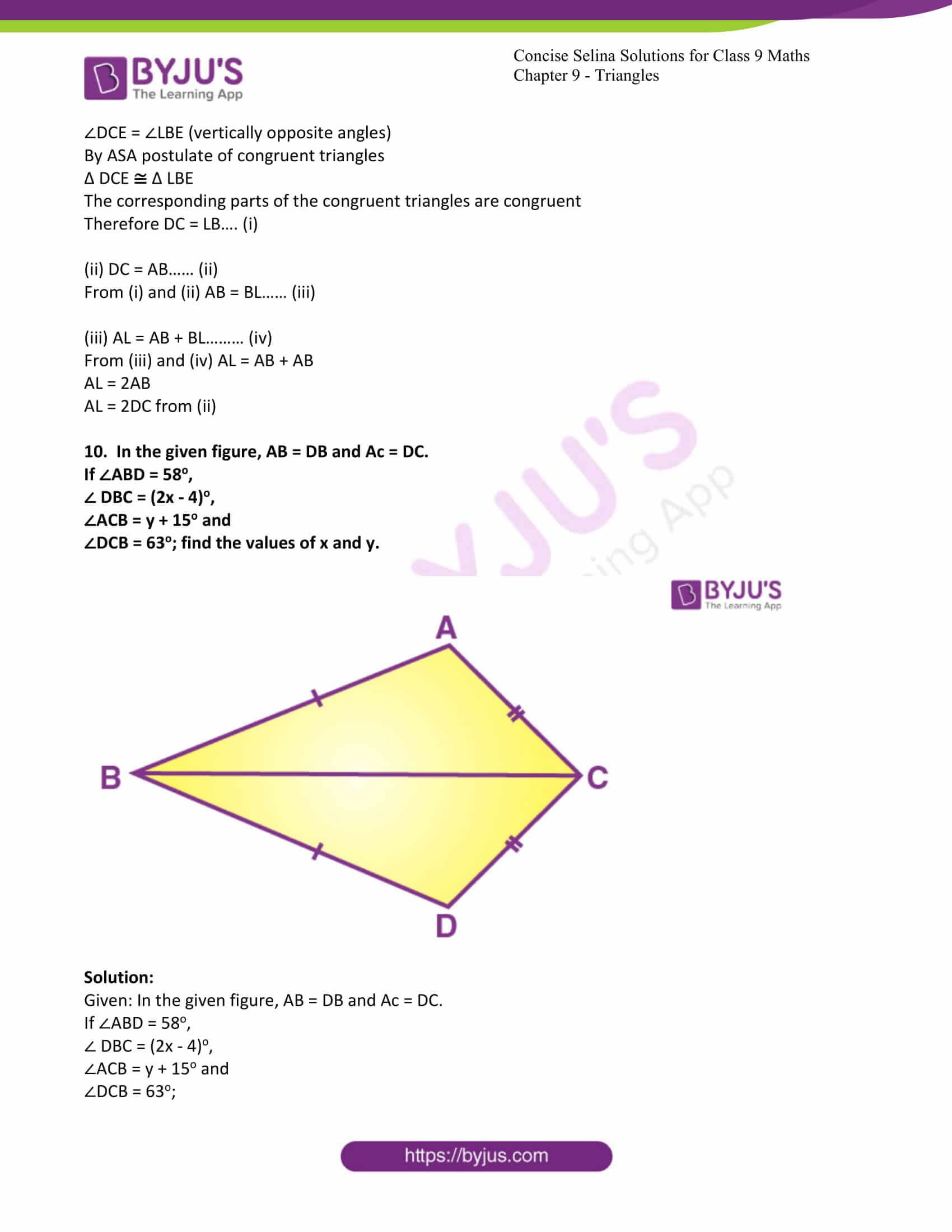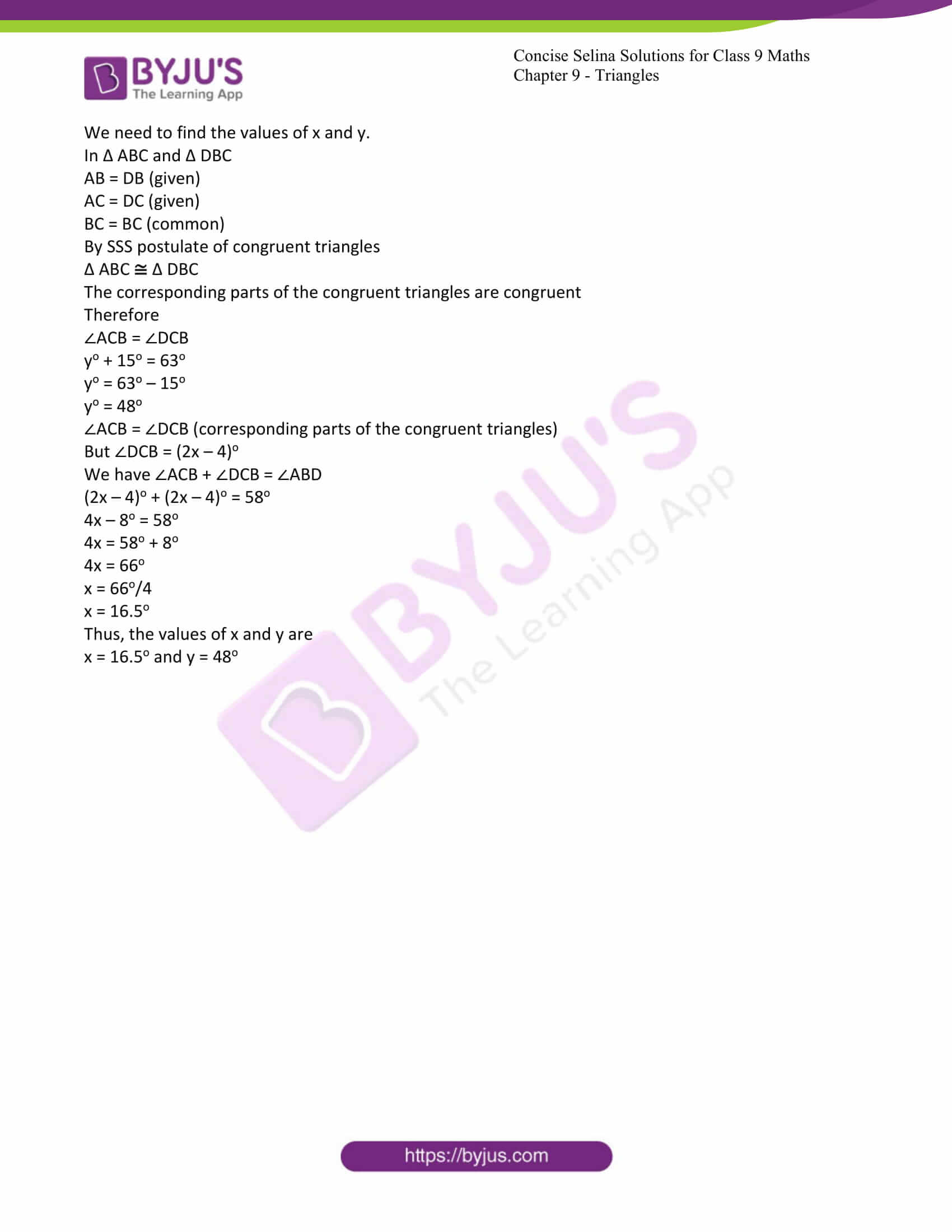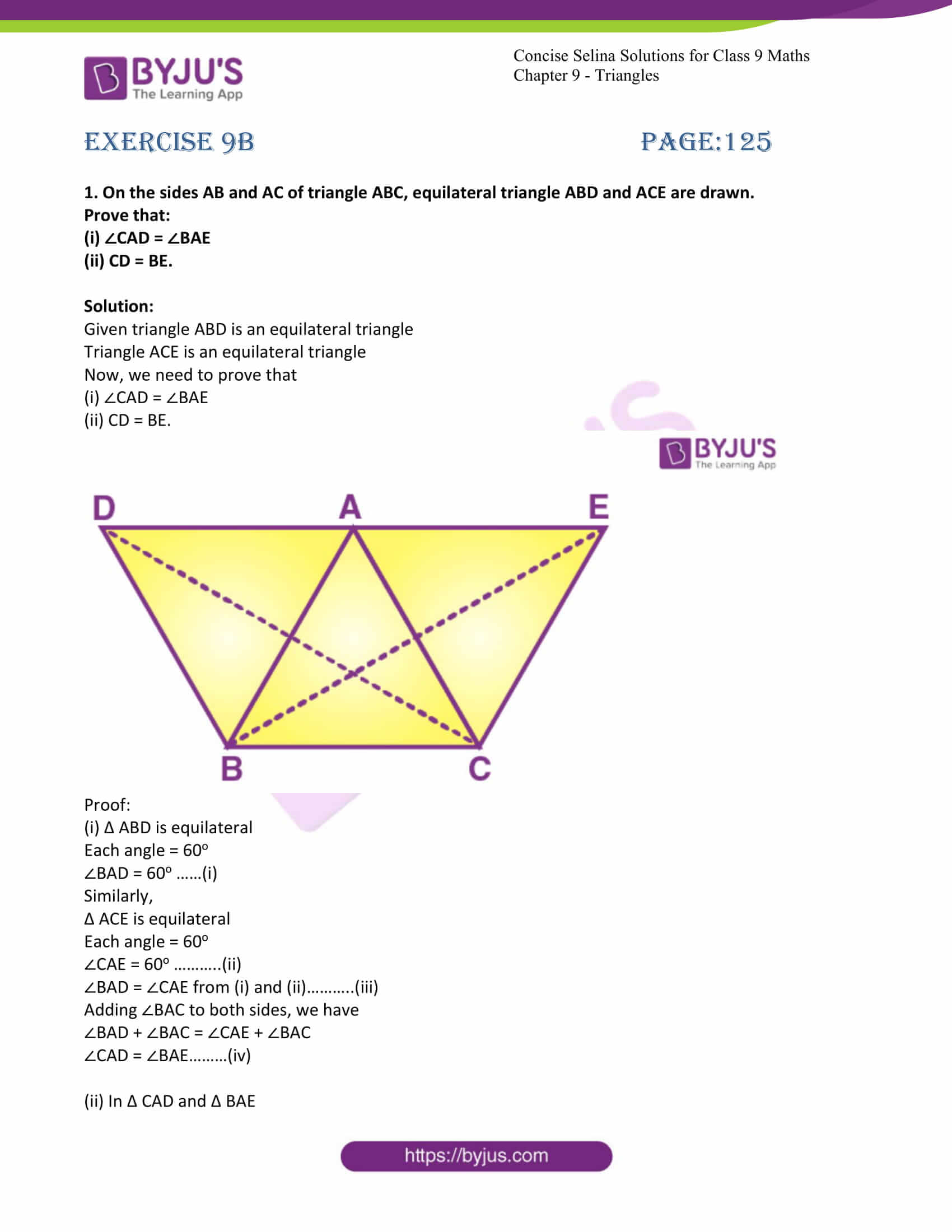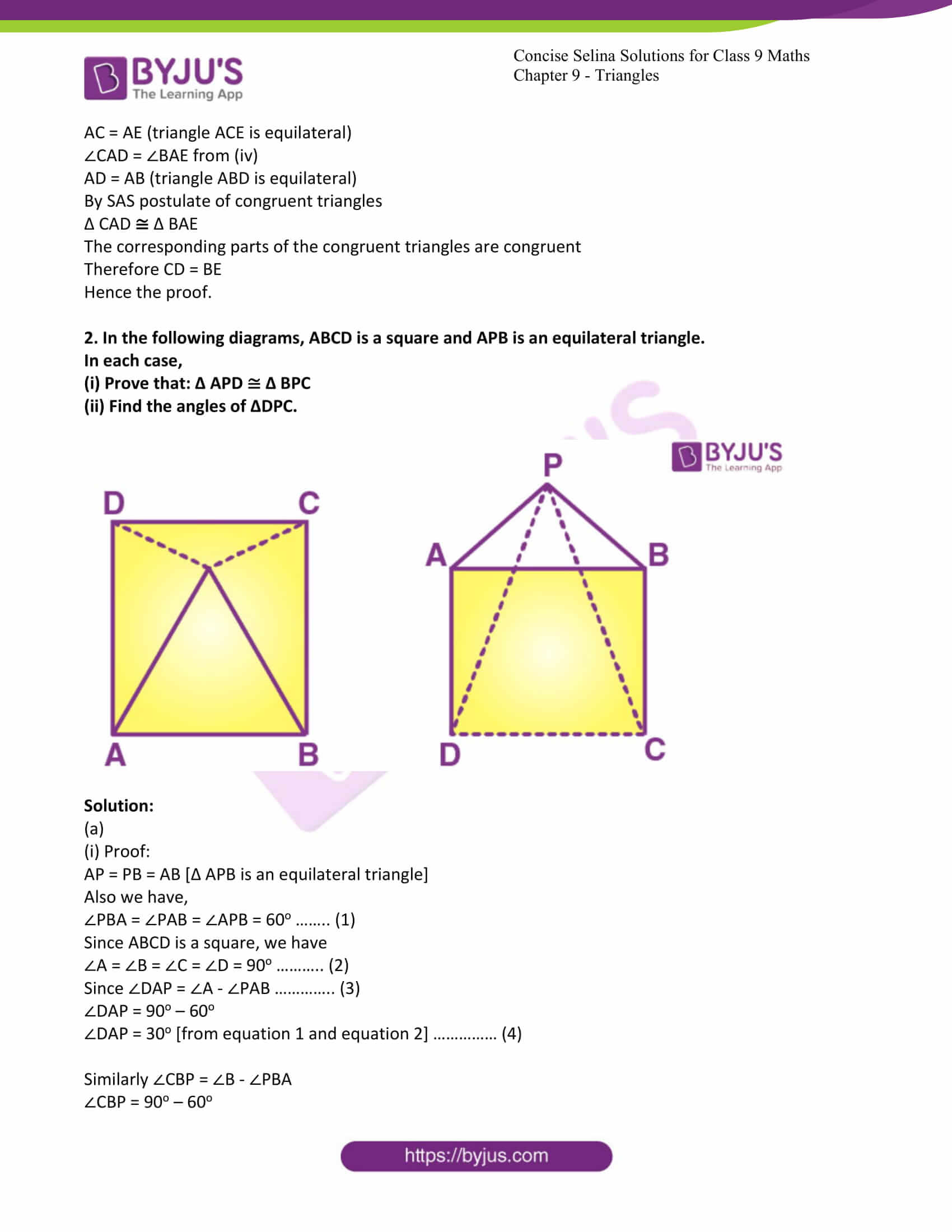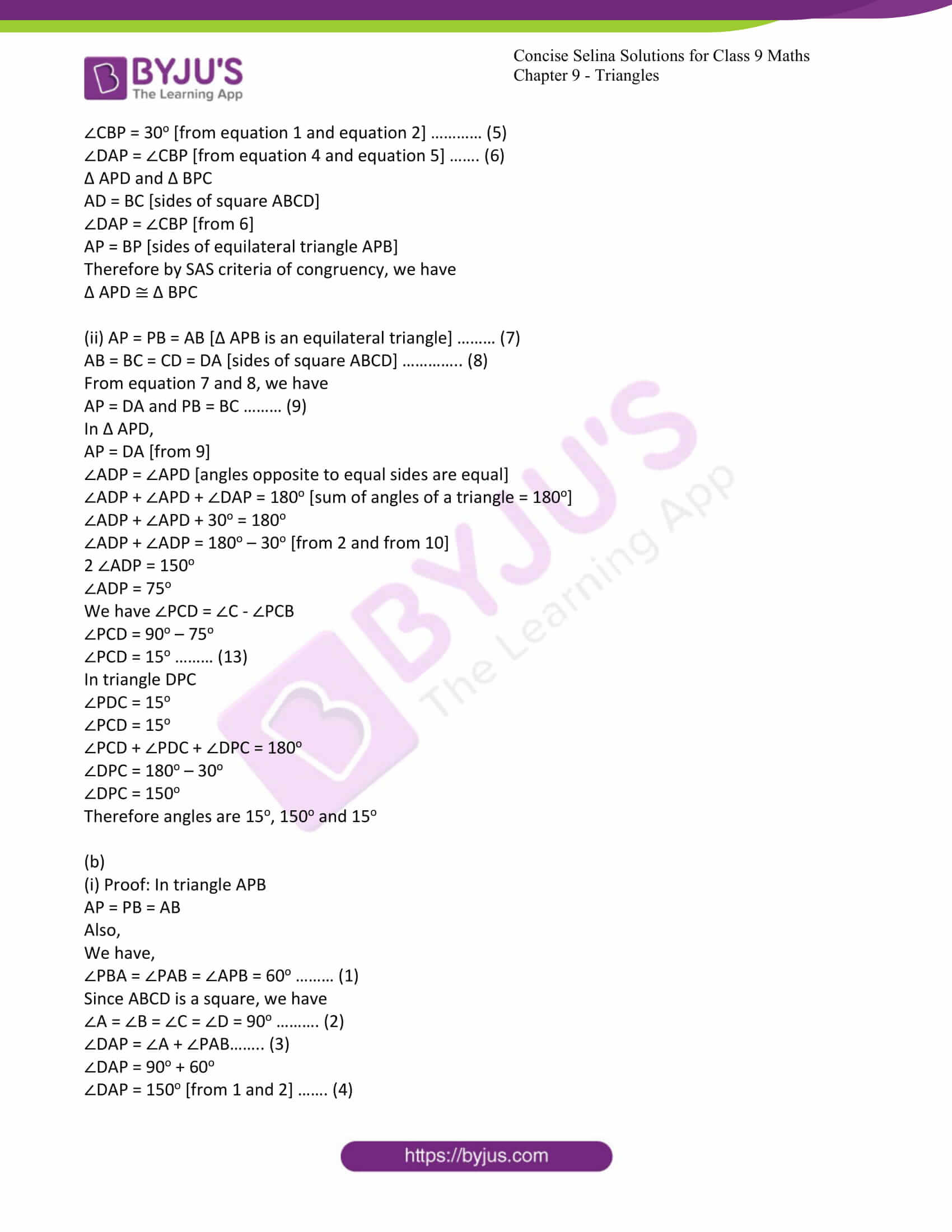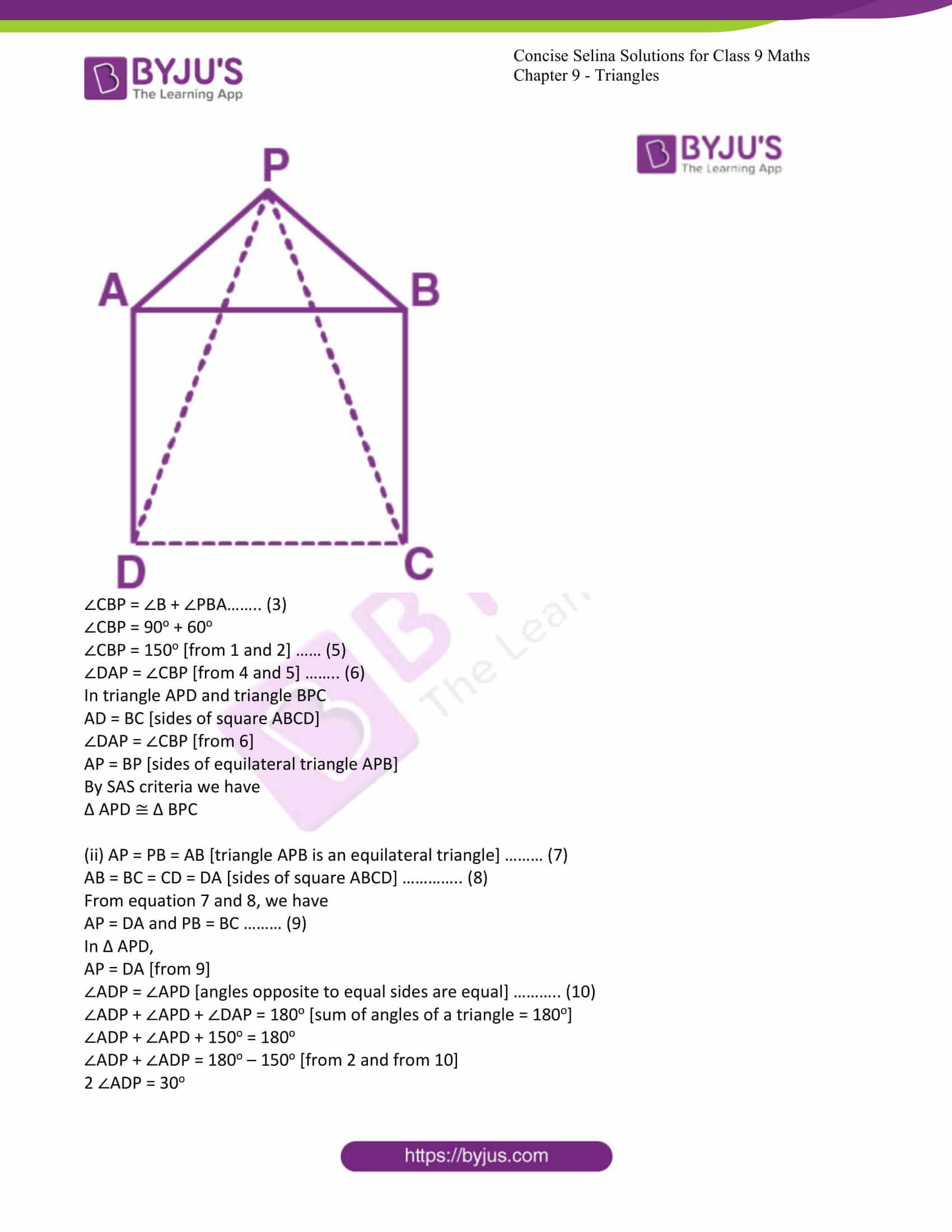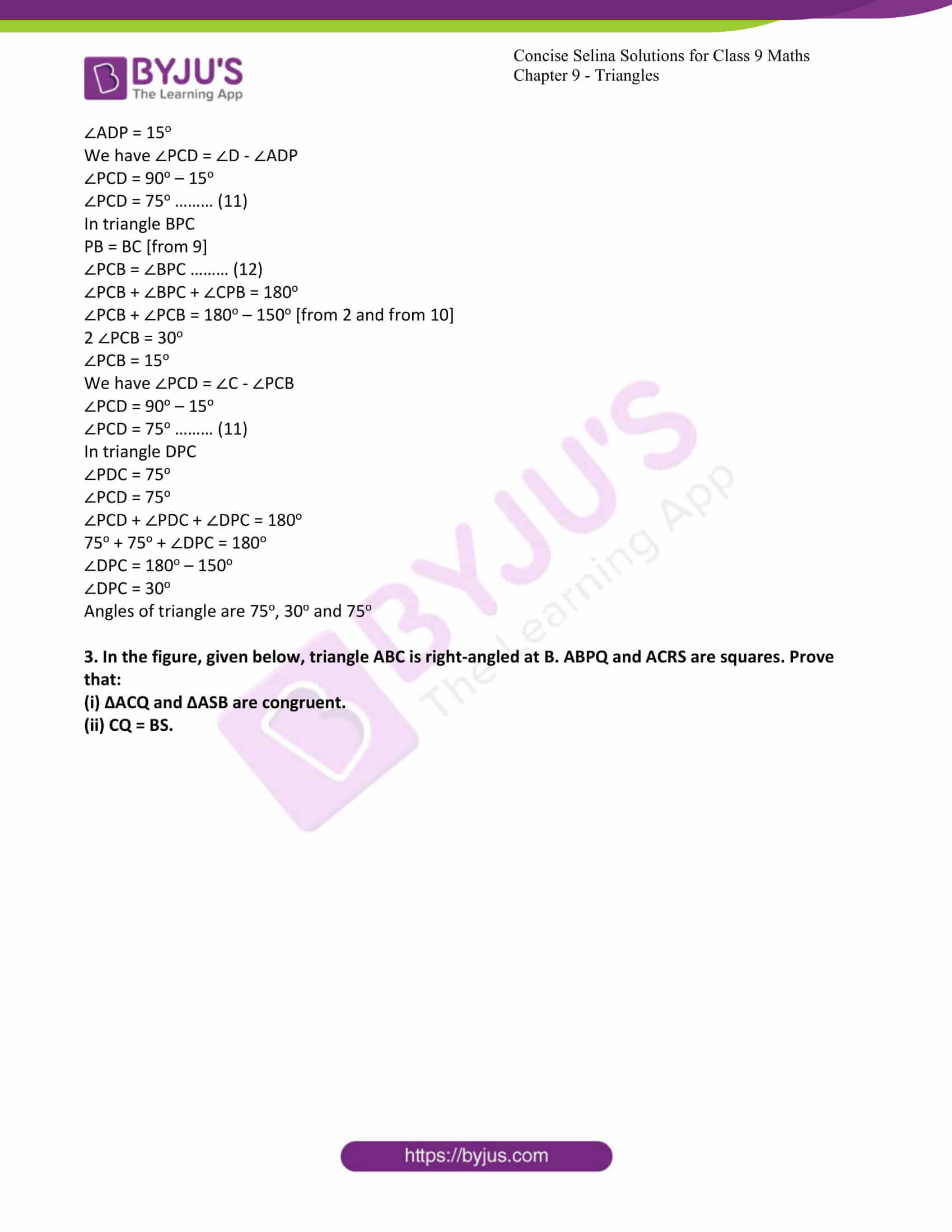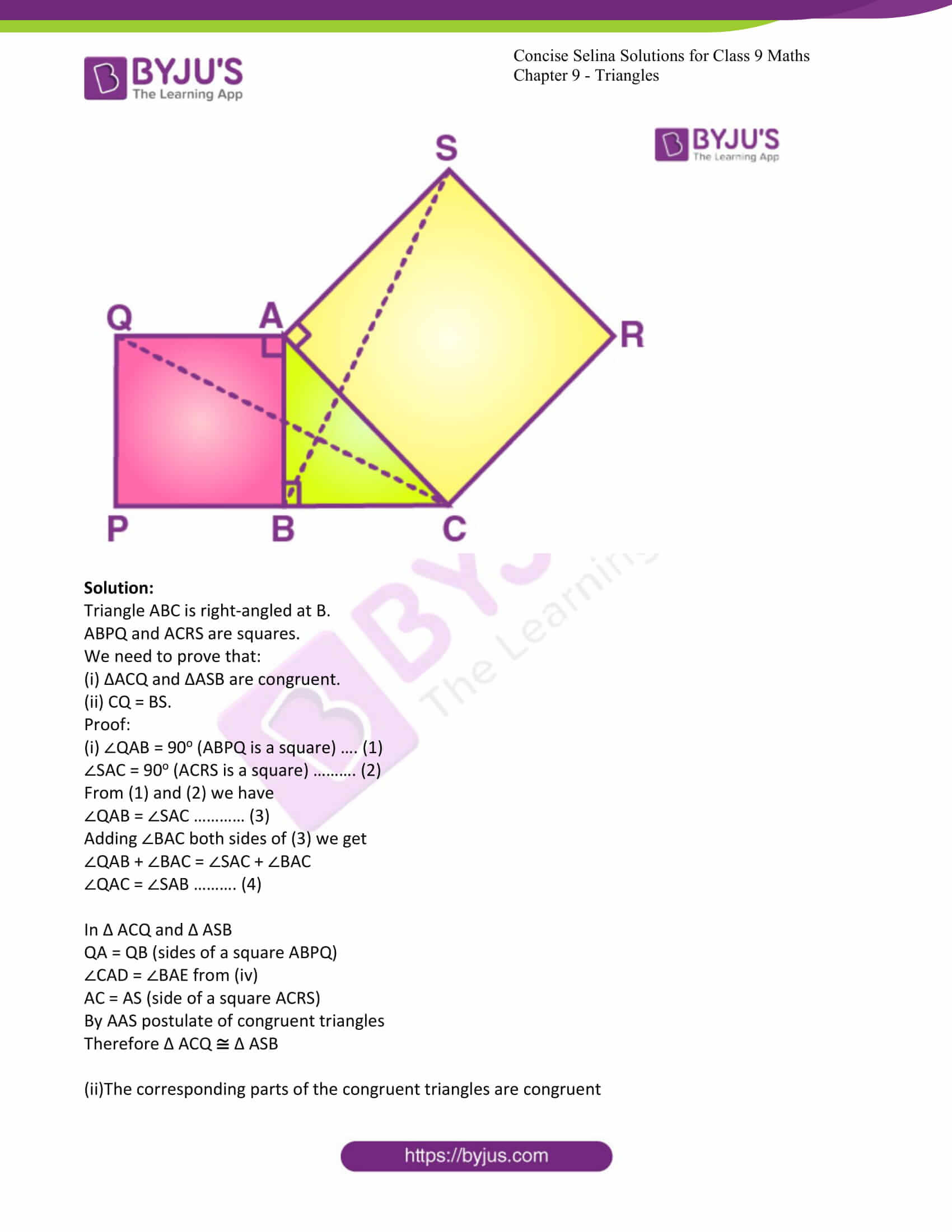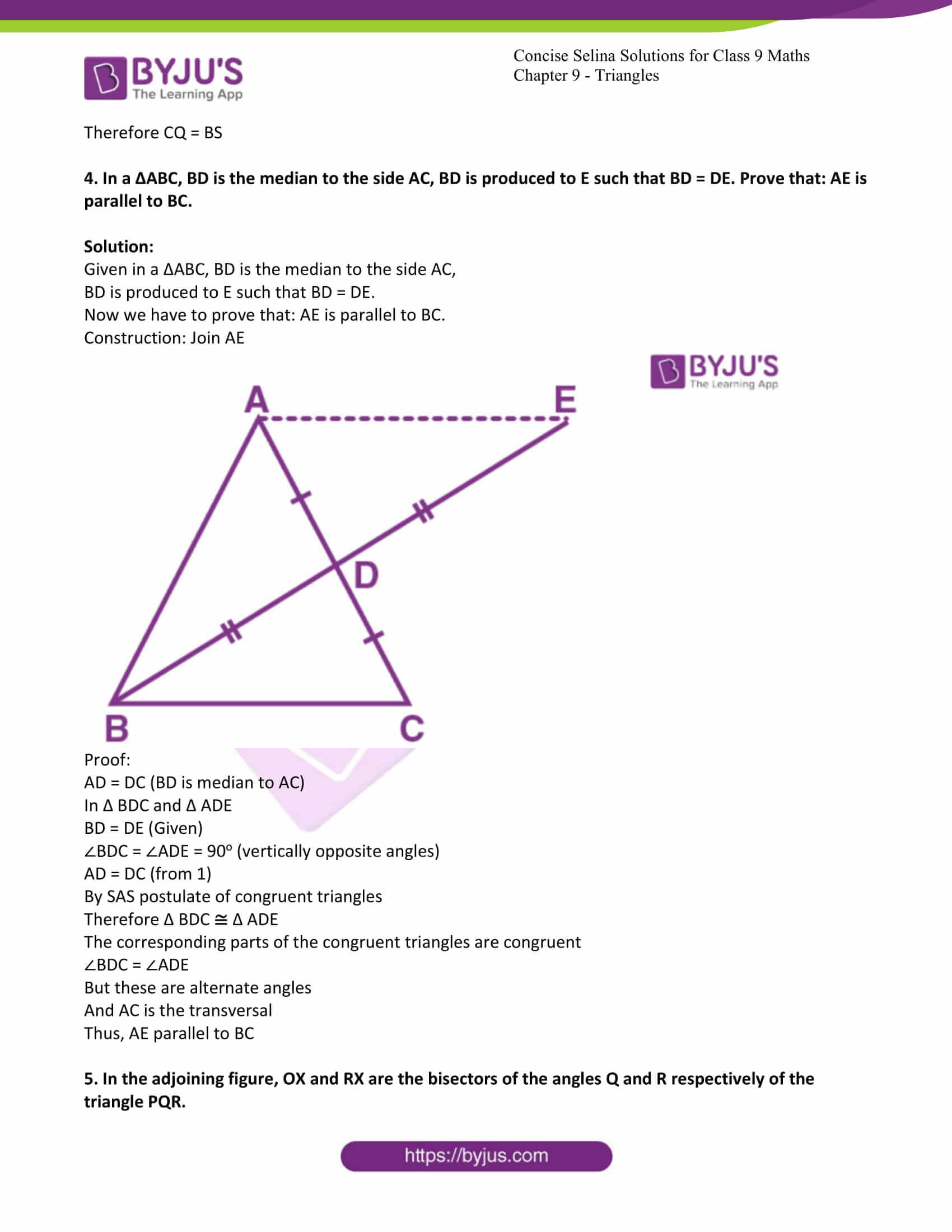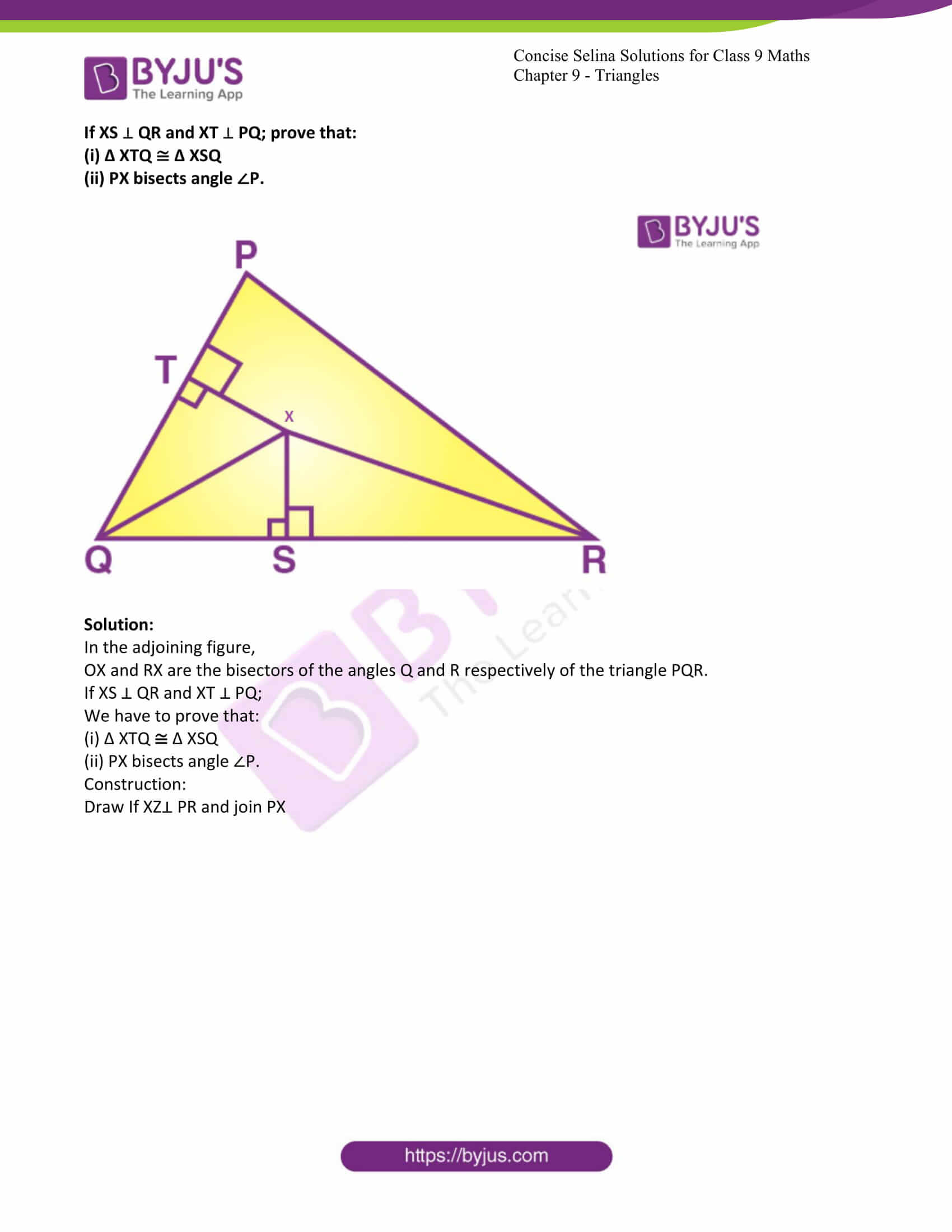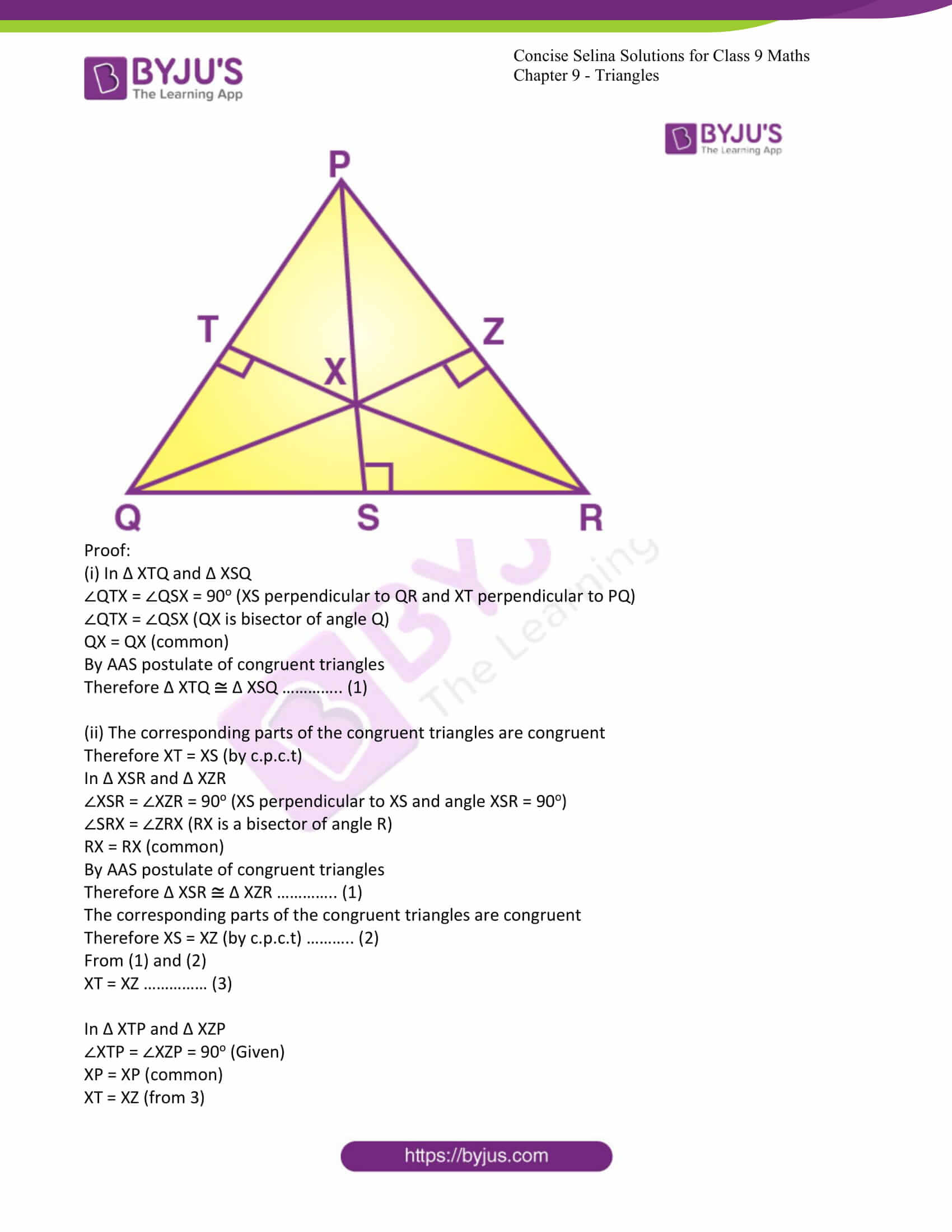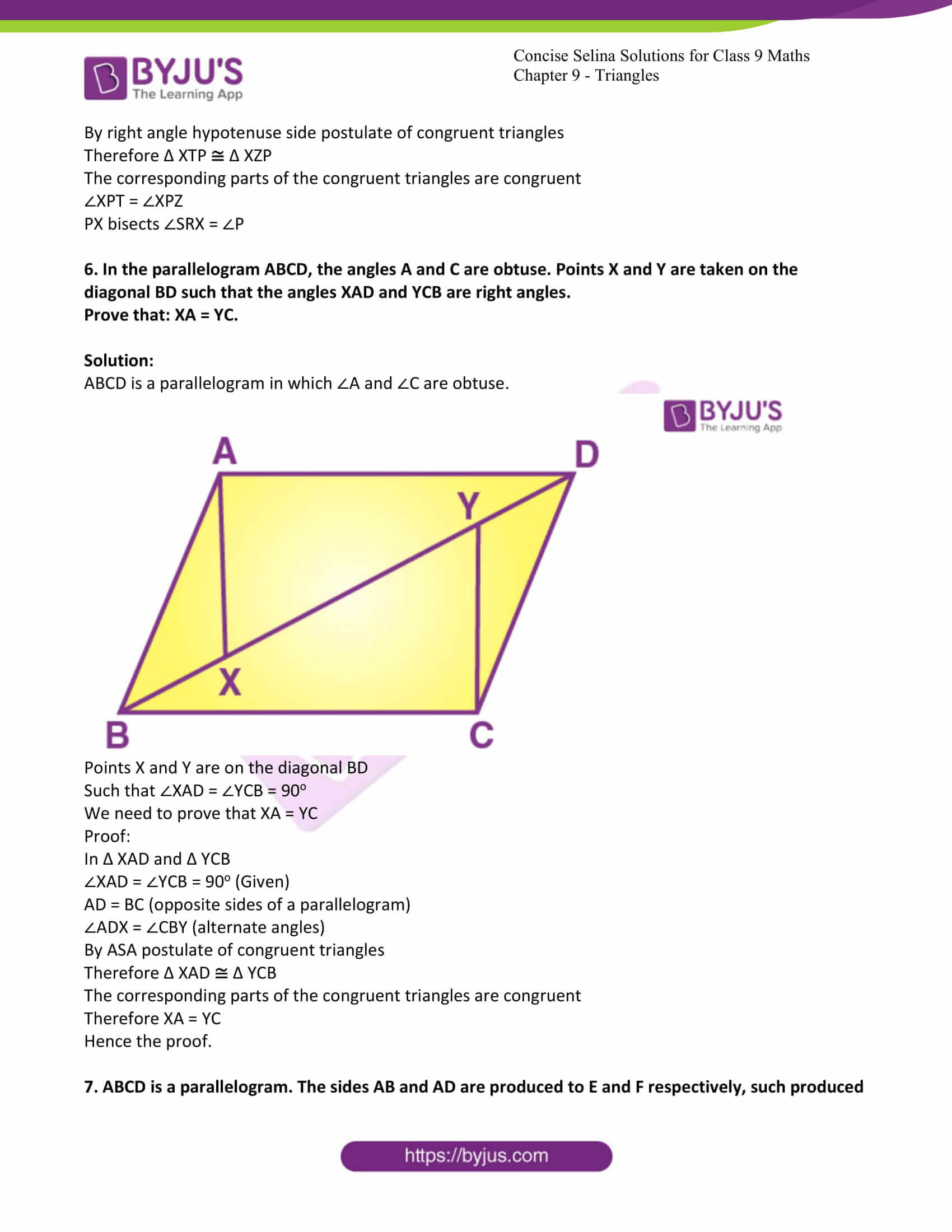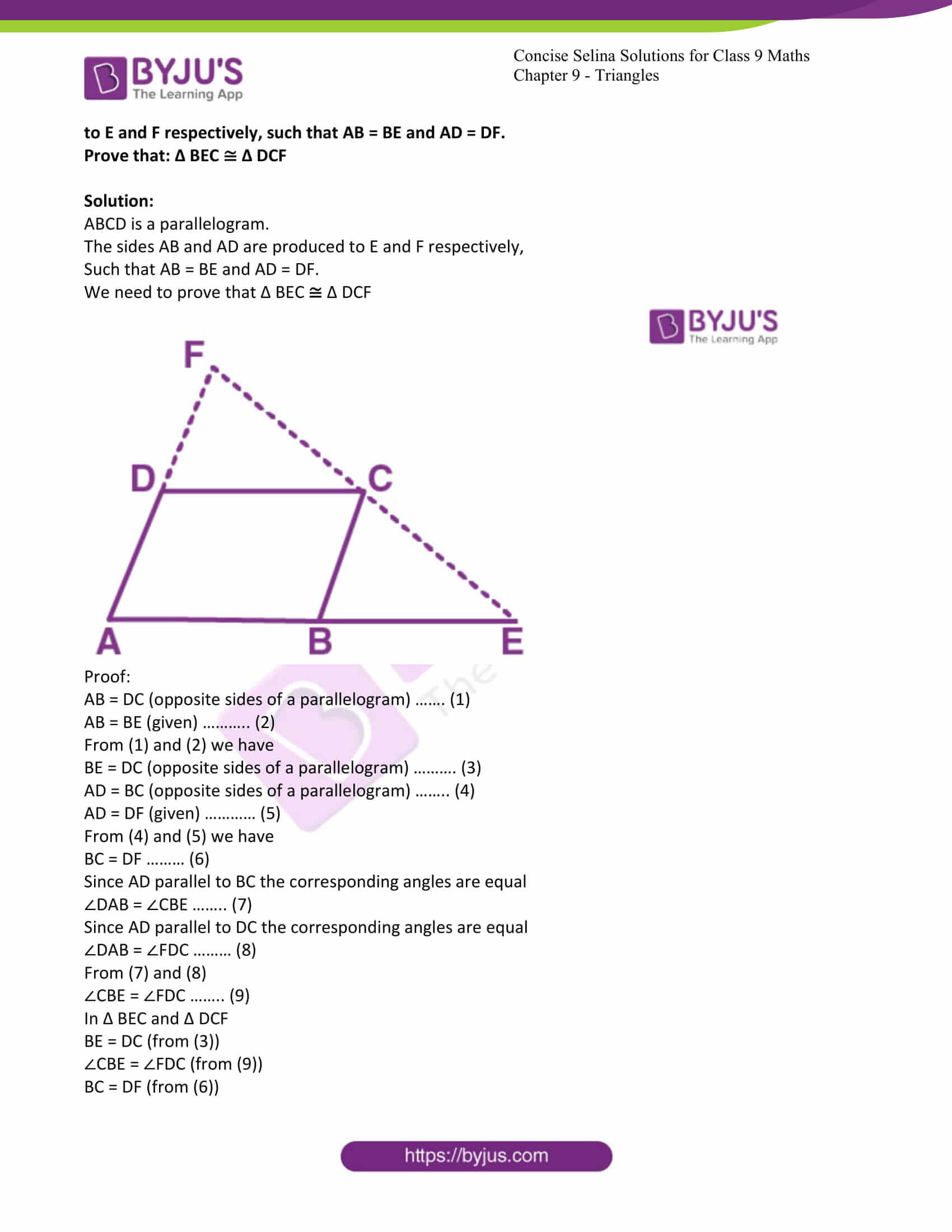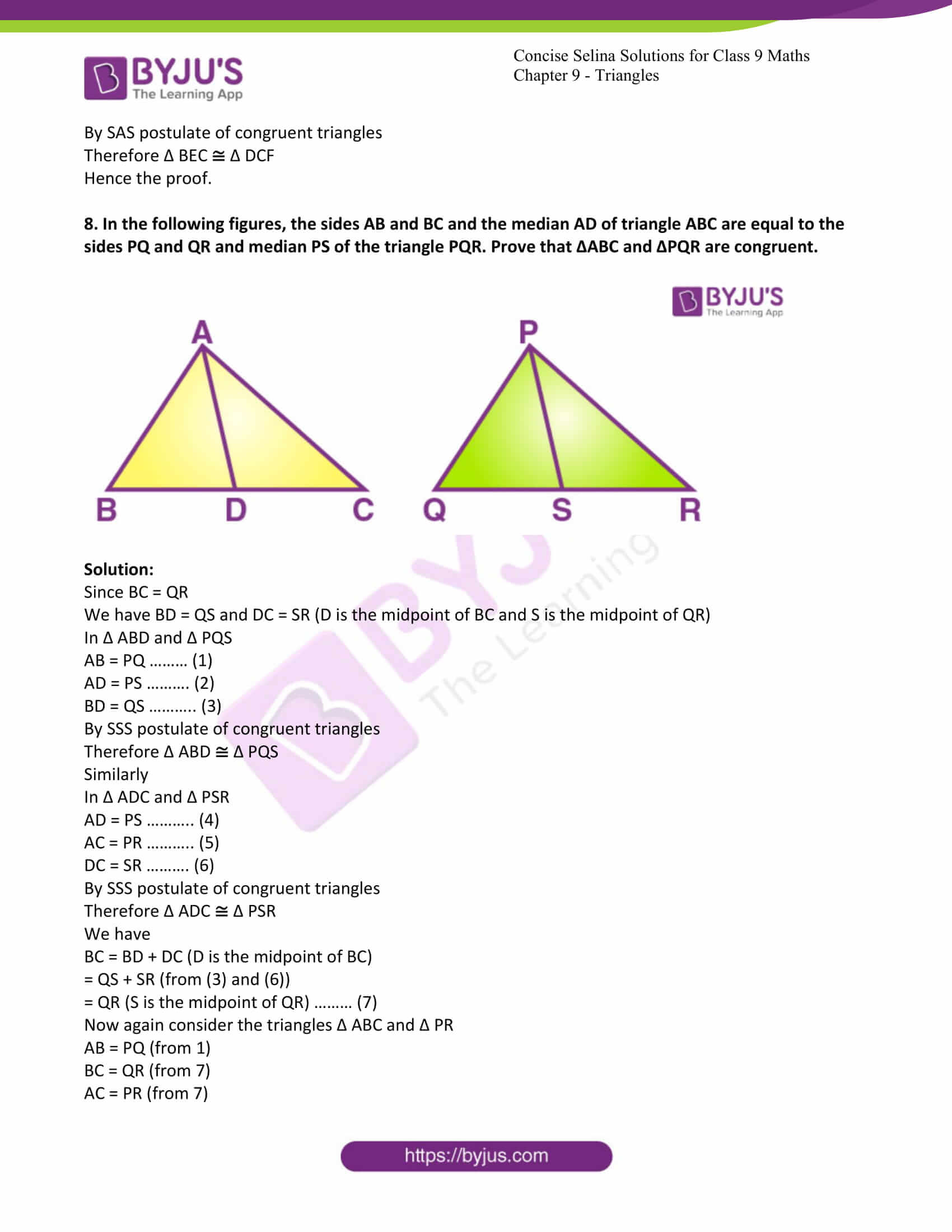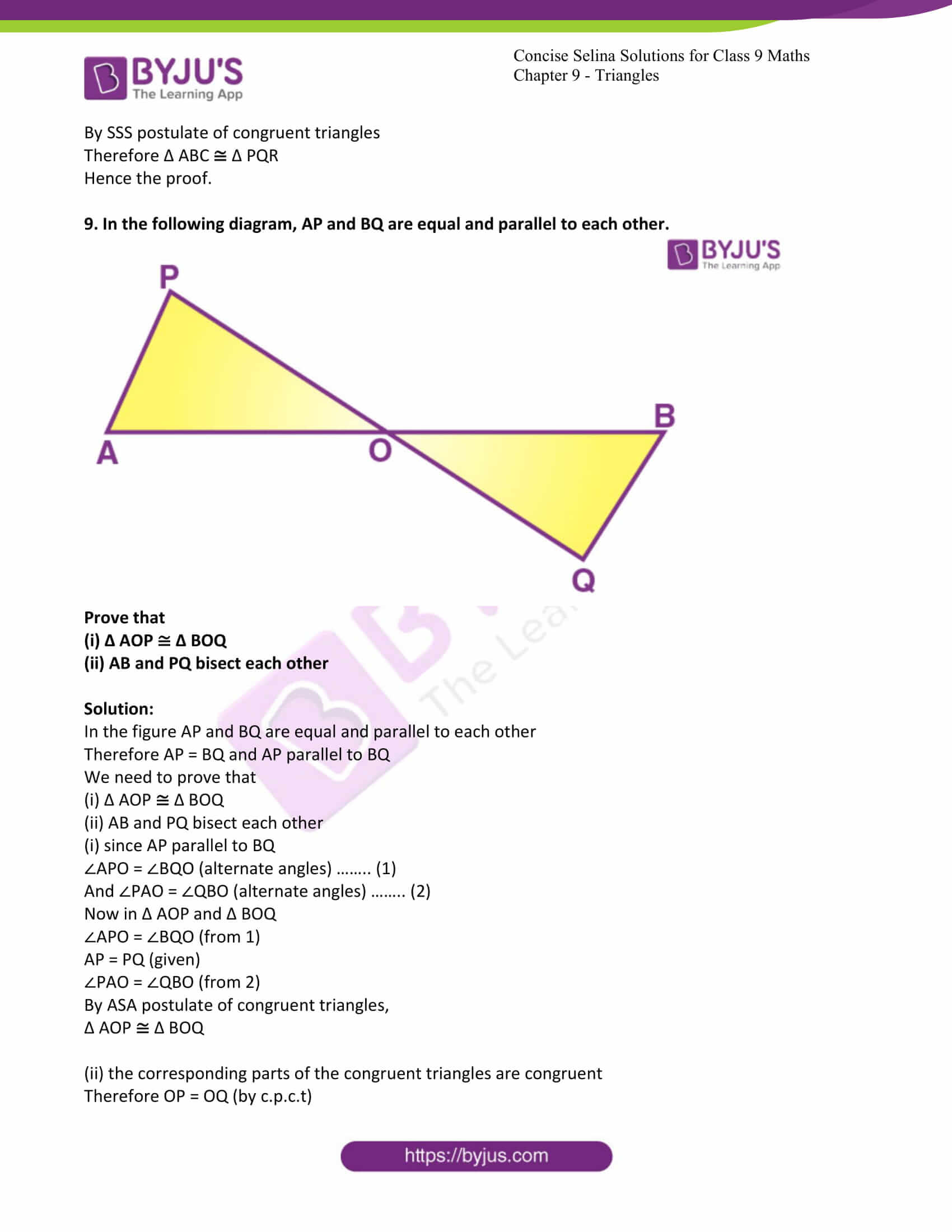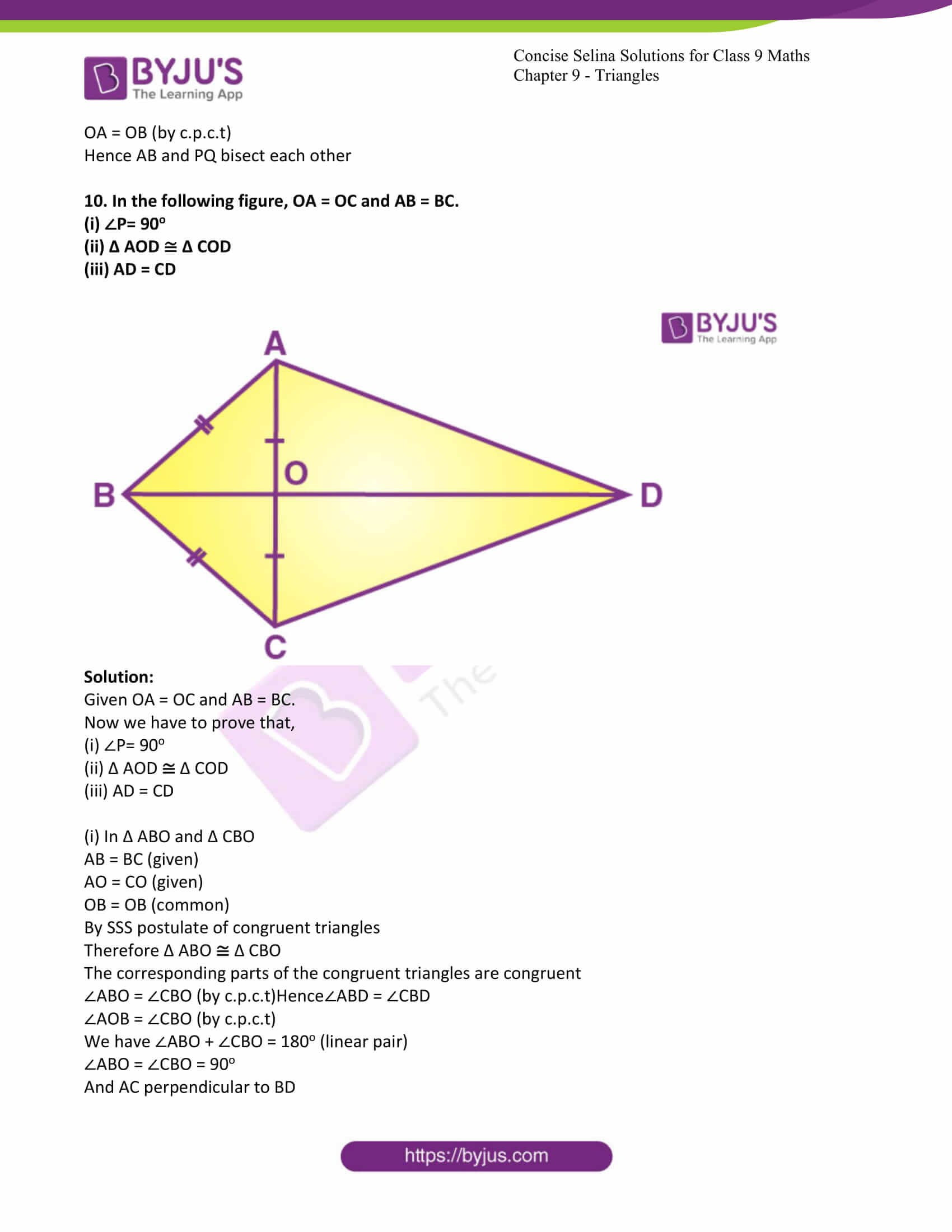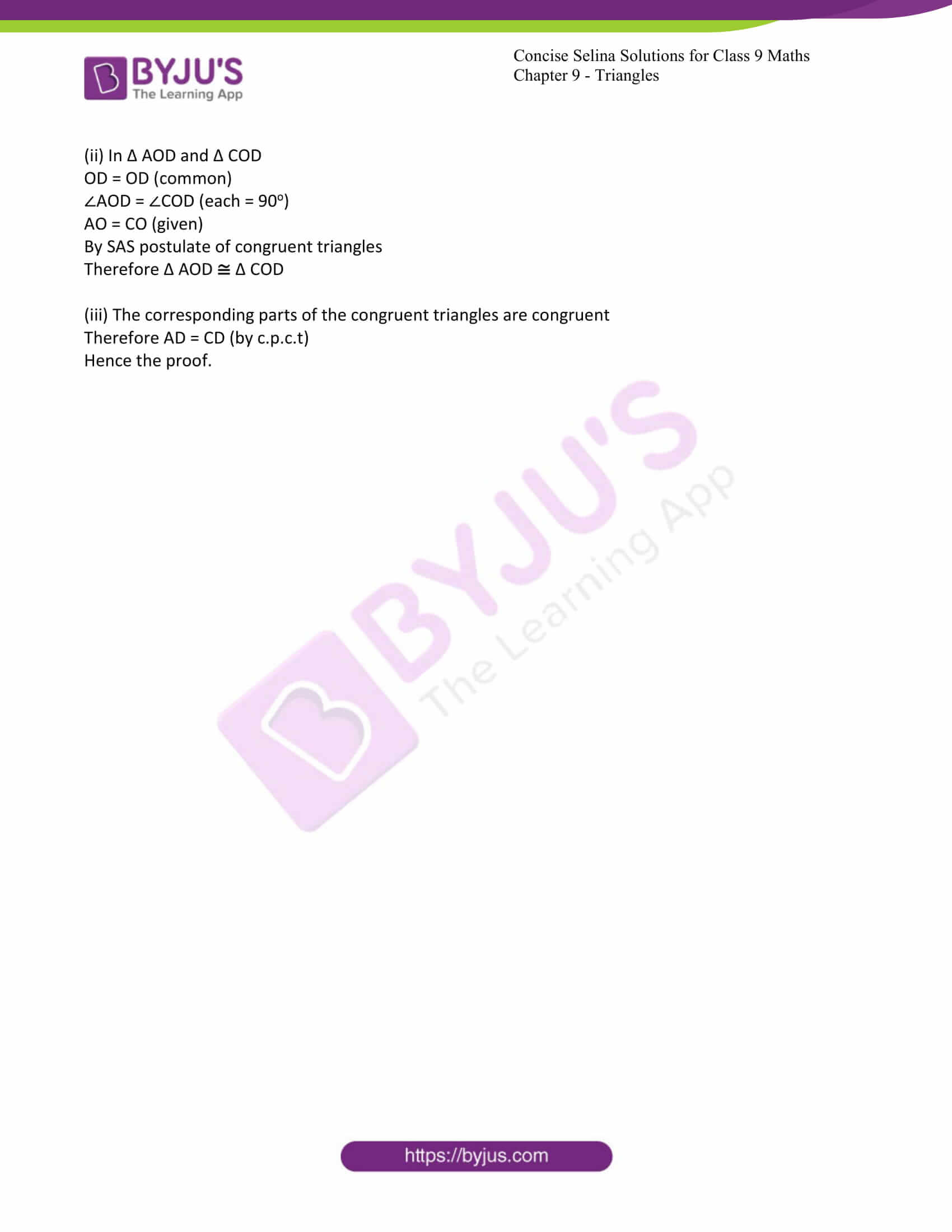Exercise 9A PAGE:103

1. Which of the following pairs of triangles are congruent? In each case, state the condition of congruency:

(a) In ∆ ABC and ∆ DEF, AB = DE, BC = EF and ∠B = ∠E.

(b) In ∆ ABC and ∆ DEF, ∠B = ∠E = 90o; AC = DF and BC = EF.

(c) In ∆ ABC and ∆ QRP, AB = QR, ∠B = ∠R and ∠C = ∠P.

(d) ∆ PQR, AB = PQ, AC = PR and BC = QR.

(e) In ∆ ABC and ∆ PQR, BC = QR, ∠A = 90o, ∠C = ∠R = 40o and ∠Q=50o.

Solution:

(a) In ∆ ABC and ∆ DEF

AB = DE (data)

BC = EF and ∠B = ∠E (given)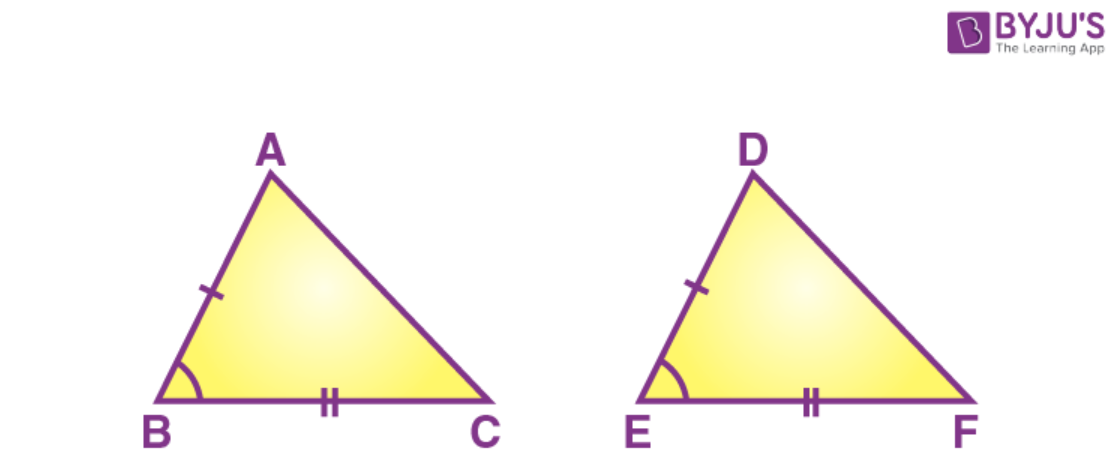By SAS criteria of congruency and given data we can conclude that,

∆ ABC and ∆ DEF are congruent to each other.

Therefore, ∆ ABC ∆ DEF.

(b) Given in ∆ ABC and ∆ DEF,

∠B = ∠E = 90o;

AC = DF

That is hypotenuse AC = hypotenuse DF

and BC = EF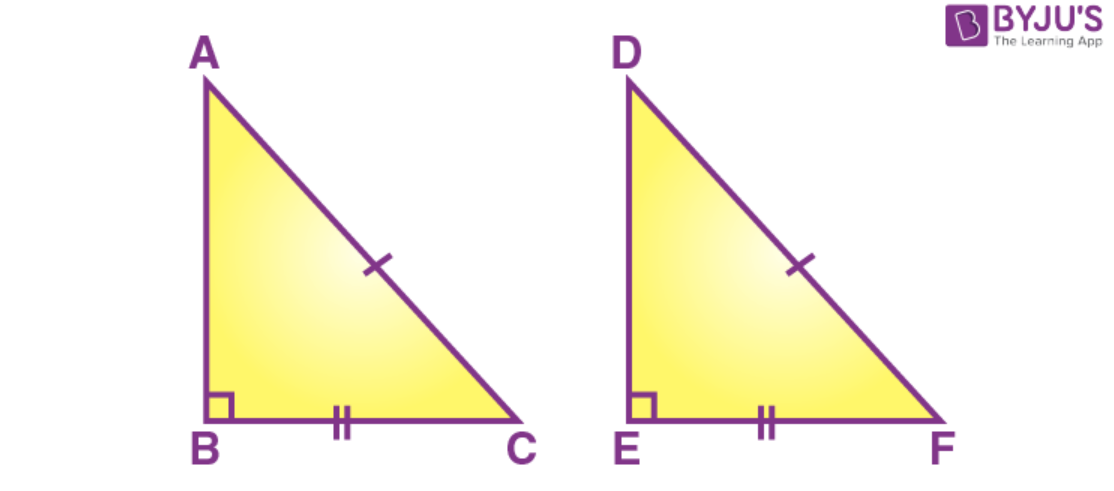By right angle hypotenuse side postulate of congruency,

The given triangles ∆ ABC and ∆ DEF are congruent to each other.

Therefore, ∆ ABC ∆ DEF.

(c) In ∆ ABC and ∆ QRP,

Data: AB = QR,

∠B = ∠R and

∠C = ∠P.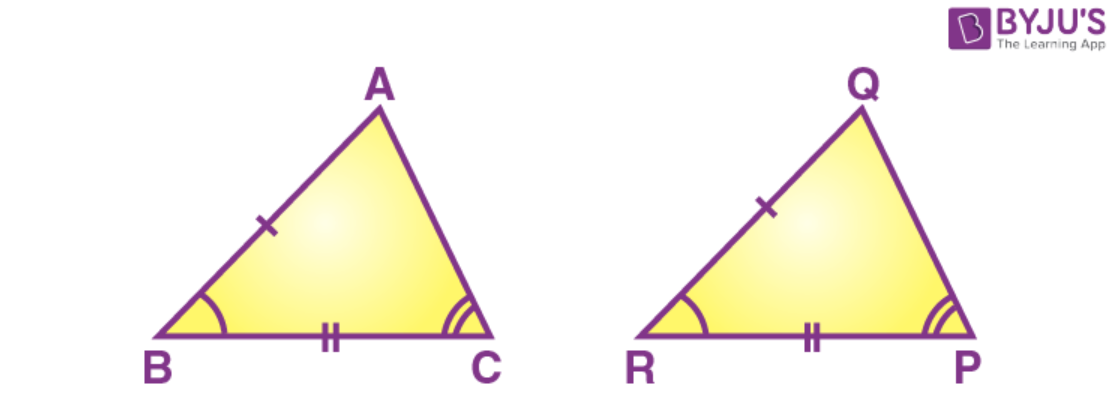By using SAS postulate and given data we can conclude that

The given triangles ∆ ABC and ∆ QRP are congruent to each other.

Therefore, ∆ ABC ∆ QRP.

(d) In In ∆ ABC and ∆ PQR,

Data: AB = PQ, AC = PR and BC = QR.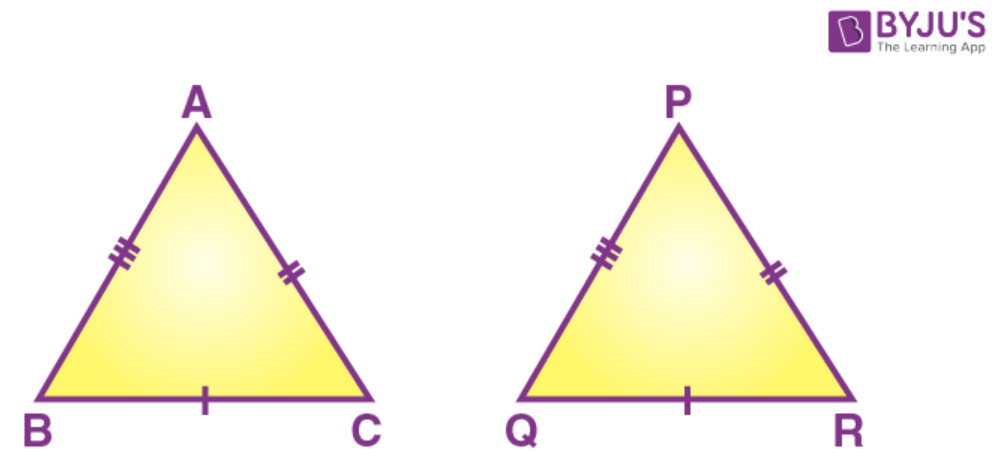By using SSS postulate of congruency and given data we can conclude that

The given triangles ∆ ABC and ∆ PQR are congruent to each other.

Therefore, ∆ ABC ∆ PQR.

(e) In ∆ ABC and ∆ PQR,

Data: BC = QR, ∠A = 90o,

∠C = ∠R = 40o and ∠Q=50o

But we know that sum of angle of triangle = 180o

Therefore, ∠P + ∠Q + ∠R = 180o

∠P + 50o + 40o = 180o

∠P + 90o = 180o

∠P = 180o – 90o

∠P = 90o

In ∆ ABC and ∆ PQR,

∠A = ∠P

∠C = ∠R

BC = QR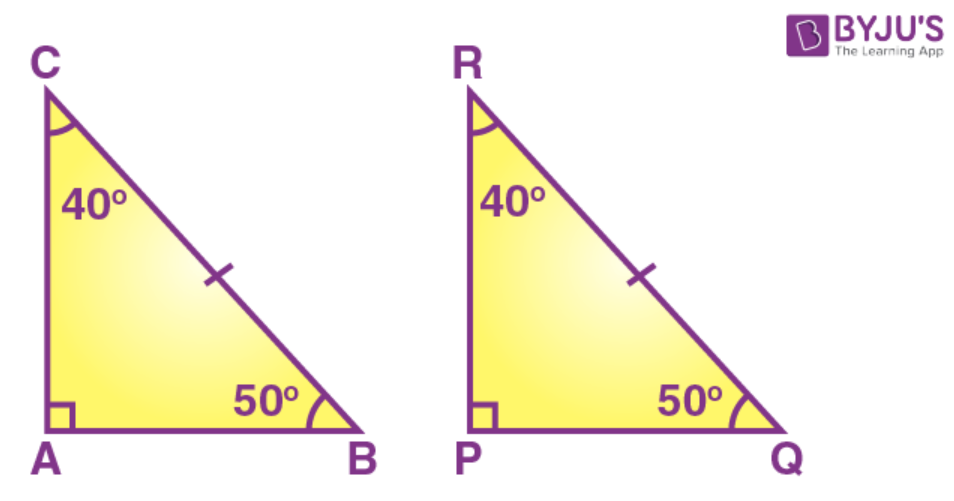By ASA postulate of congruency,

The given triangles ∆ ABC and ∆ PQR are congruent to each other.

Therefore, ∆ ABC ∆ PQR.

2. The given figure shows a circle with centre O. P is mid-point of chord AB.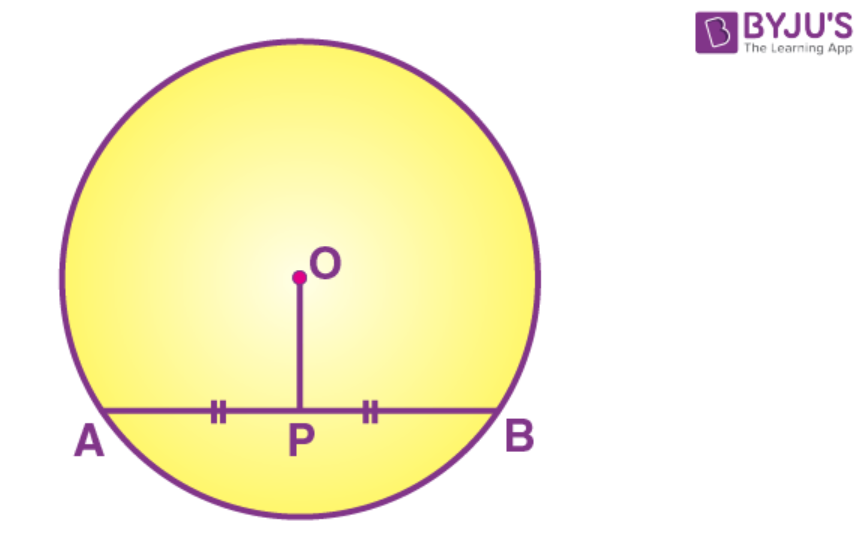Show that OP is perpendicular to AB.

Solution:

Data: in the given figure O centre of circle.

P is mid-point of chord AB. AB is a chord

P is a point on AB such that AP = PB

Now we have to prove that OP ⊥ AB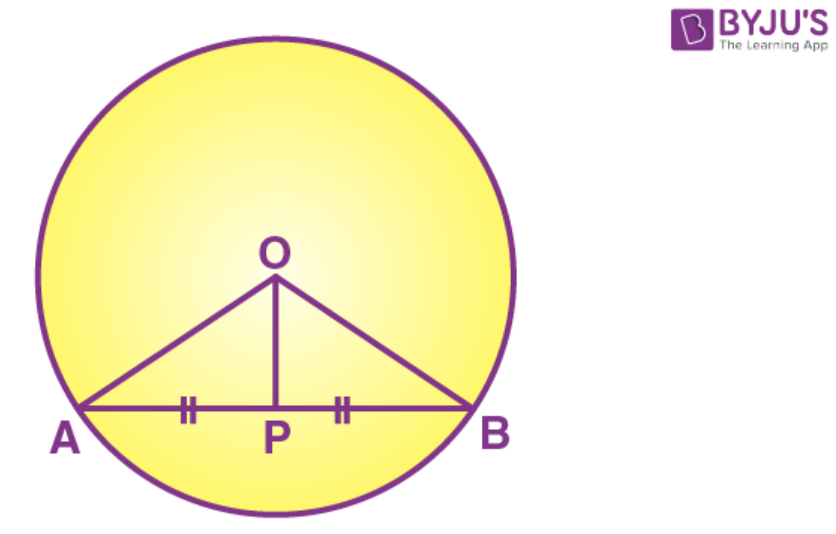Construction: Join OA and OB

Proof: In ∆ OAP and ∆ OBP

OA = OB (because radii of common circle)

OP = OP (common)

AP = PB (data)

By SSS postulate of congruent triangles

The given triangles ∆ OAP and ∆ OBP are congruent to each other.

Therefore, ∆ OAP ∆ OBP.

The corresponding parts of the congruent triangles are congruent.

∠OPA = ∠OPB (by Corresponding parts of Congruent triangles)

But ∠OPA + ∠OPB = 180o (linear pair)

∠OPA = ∠OPB = 90o

Hence OP ⊥ AB

3. The following figure shows a circle with centre O.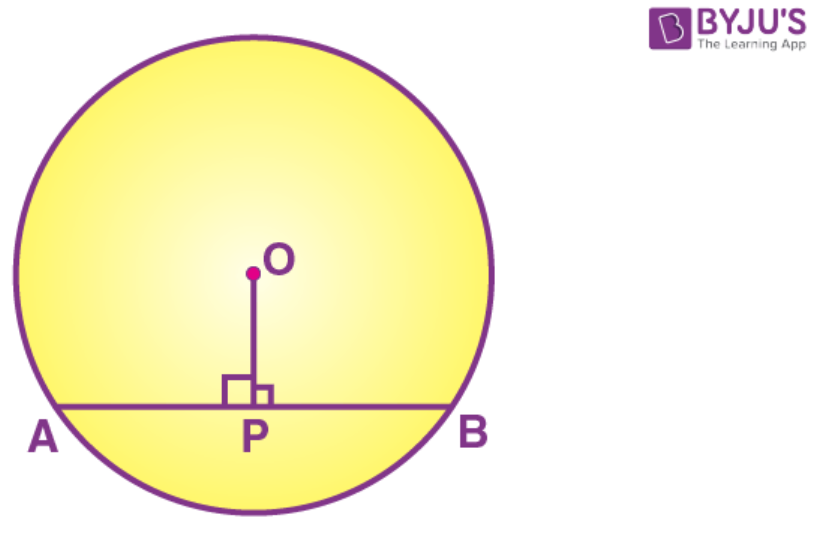If OP is perpendicular to AB, prove that AP=BP.

Solution:

Given: In the figure, O is the centre of the circle,

And AB is chord. P is a midpoint on AB such that AP = PB

We need to prove that AP = BP,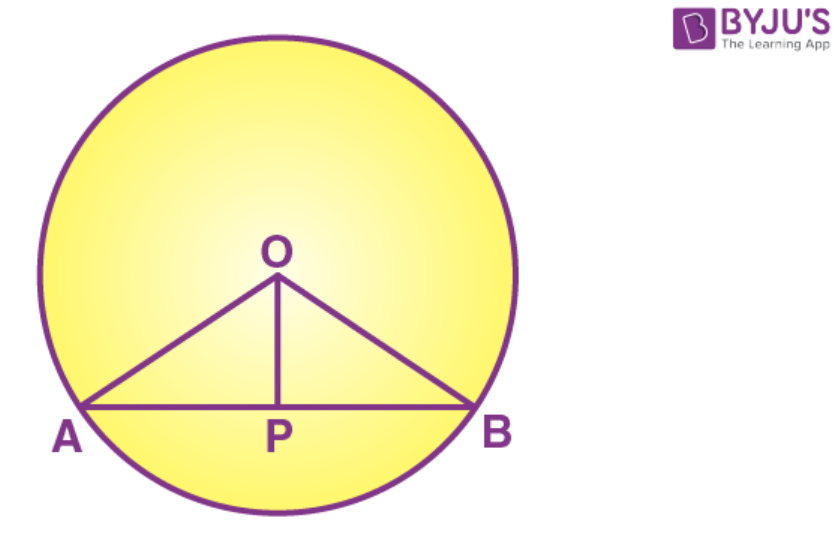Construction: Join OA and OB

Proof: In right angle triangles ∆ OAP and ∆ OBP

Hypotenuse OA = Hypotenuse OB (because radii of common circle)

Side OP = OP (common)

AP = PB (data)

By SSS postulate of congruent triangles

The given triangles ∆ OAP and ∆ OBP are congruent to each other.

Therefore, ∆ OAP ∆ OBP.

The corresponding parts of the congruent triangles are congruent.

AP = BP (by Corresponding parts of Congruent triangles)

Hence the proof.

4. In a triangle ABC, D is mid-point of BC; AD is produced up to E so that DE = AD. Prove that: (i) ∆ ABD and ∆ ECD are congruent.

(ii) AB=EC

(iii) AB is parallel to EC.

Solution:

Given ∆ ABC in which D is the mid-point of BC

We need to prove that

(i) ∆ ABD ∆ ECD

(ii) AB = EC

(iii) AB ∥ EC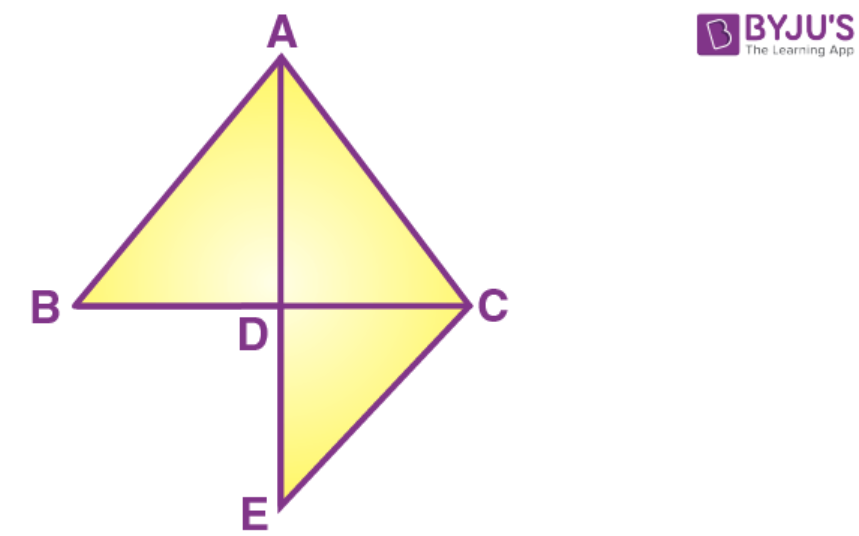(i) In ∆ ABD and ∆ ECD

BD = DC (D is the midpoint of BC)

∠ADB = ∠CDE (vertically opposite angles)

By SAS postulate of congruency of triangles, we have

∆ ABD ∆ ECD

(ii) The corresponding parts of congruent triangles are congruent

Therefore, AB = EC (corresponding parts of congruent triangles)

(iii) Also, we have ∠DAB = ∠DEC (corresponding parts of congruent triangles)

AB ∥ EC [∠DAB = ∠DEC are alternate angles]

5. A triangle ABC has ∠B = ∠C. Prove that:

(i) The perpendiculars from the mid-point of BC to AB and AC are equal.

(ii) The perpendiculars form B and C to the opposite sides are equal.

Solution:

(i) Given ∆ ABC in which ∠B = ∠C.

DL is perpendicular from D to AB

DM is the perpendicular from D to AC.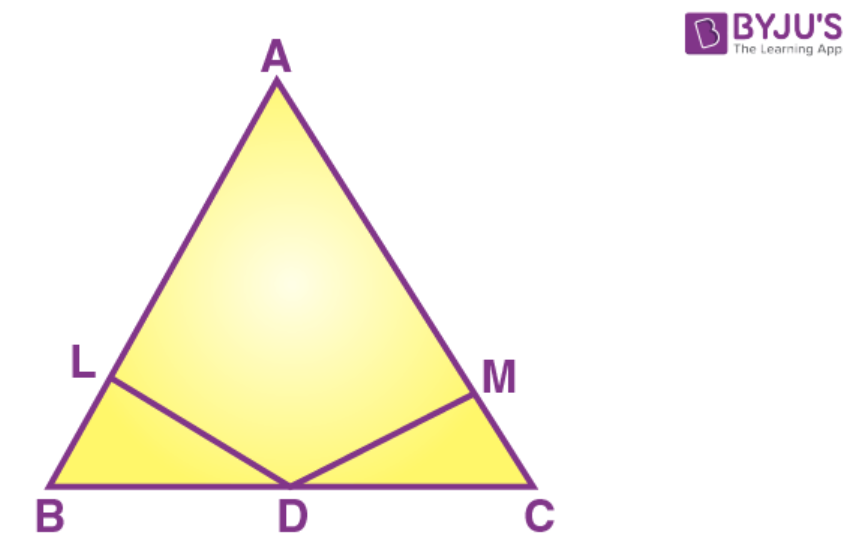We need to prove that

DL = DM

Proof:

In ∆ ABC and ∆ DMC (DL perpendicular to AB and DM perpendicular to AC)

∠DLB = ∠DMC = 90o

∠B = ∠C (Given)

BD = DC (D is midpoint of BC)

By AAS postulate of congruent triangles

∆ DLB ∆ DMC

The corresponding parts of the congruent triangles are congruent

Therefore DL = DM

(ii) Given ∆ ABC in which ∠B = ∠C.

BP is perpendicular from D to AC

CQ is the perpendicular from C to AB.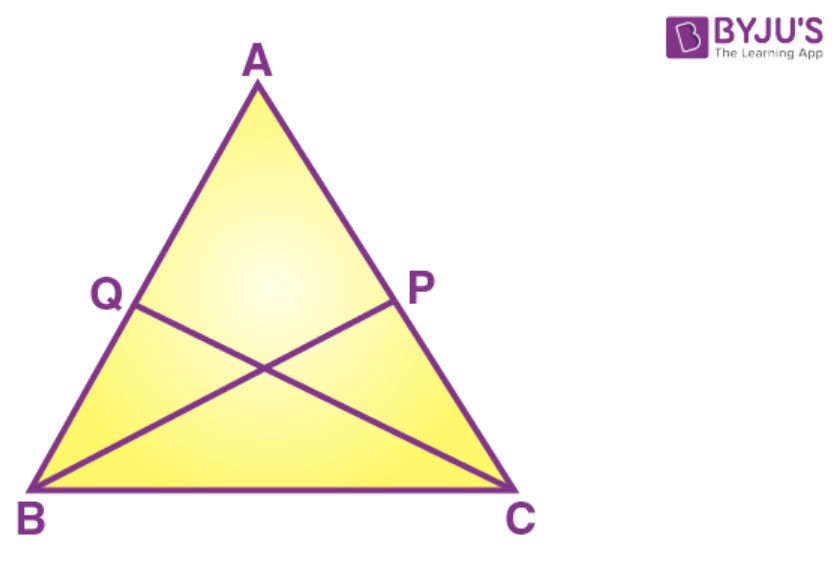We need to prove that

BP = CQ

Proof:

In ∆ BPC and ∆ CQB (BP perpendicular to AC and CQ perpendicular to AB)

∠BPC = ∠CQB = 90o

∠B = ∠C (Given)

BC = BC (common)

By AAS postulate of congruent triangles

∆ BPC ∆ CQB

The corresponding parts of the congruent triangles are congruent

Therefore BP = CQ

6. The perpendicular bisector of the sides of a triangle AB meet at I. Prove that: IA = IB = IC

Solution:

Given triangle ABC in which AD is the perpendicular bisector of BC

BE is the perpendicular bisector of CA

CF is the perpendicular bisector of AB

AD, BE and CF meet at I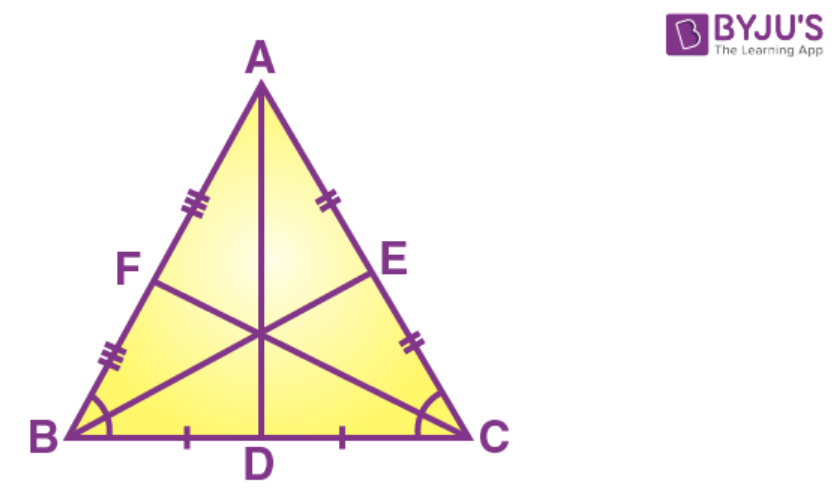We need to prove that

IA = IB = IC

Proof:

In ∆ BID and ∆ CID

BD = DC (given)

∠BDI = ∠CDI = 90o (AD is perpendicular bisector of BC)

BC = BC (common)

By SAS postulate of congruent triangles

∆ BID ∆ CID

The corresponding parts of the congruent triangles are congruent

Therefore IB = IC

Similarly, In ∆ CIE and ∆ AIE

CE = AE (given)

∠CEI = ∠AEI = 90o (AD is perpendicular bisector of BC)

IE = IE (common)

By SAS postulate of congruent triangles

∆ CIE ∆ AIE

The corresponding parts of the congruent triangles are congruent

Therefore IC = IA

Thus, IA = IB = IC

7. A line segment AB is bisected at point P and through point P another line segment PQ, which is perpendicular to AB, is drawn. Show that: QA = QB.

Solution:

Given triangle ABC in which AB is bisected at P

PQ is the perpendicular to AB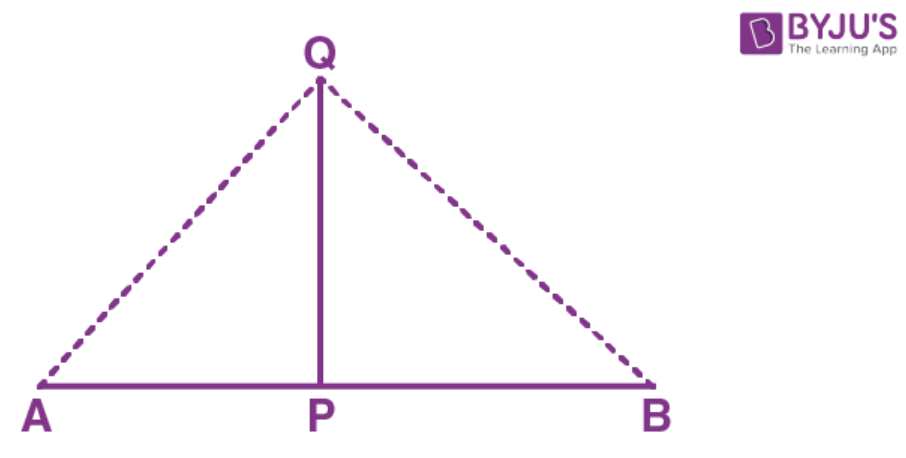We need to prove that

QA = QB

Proof:

In ∆ APQ and ∆ BPQ

AP = PB (P is the midpoint of AB)

∠APQ = ∠BPQ = 90o (PQ is perpendicular to AB)

BC = BC (common)

By SAS postulate of congruent triangles

∆ APQ ∆ BPQ

The corresponding parts of the congruent triangles are congruent

Therefore QA = QB

8. If AP bisects angle BAC and M is any point on AP, prove that the perpendiculars drawn from M to AB and AC are equal.

Solution:

From M, draw ML such that ML is perpendicular to AB and MN is perpendicular to AC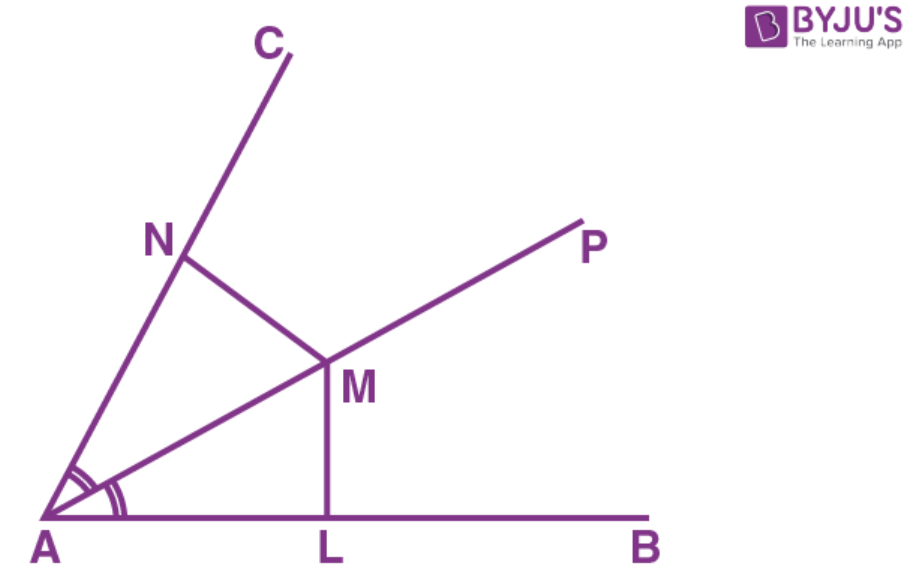In ∆ ALM and ∆ ANM

∠LAM = ∠MAN (AP is the bisector of ∠BAC)

∠ALM = ∠ANM = 90o (ML is perpendicular to AB and MN is perpendicular to AC)

AM = AM (common)

By AAS postulate of congruent triangles

∆ ALM ∆ ANM

The corresponding parts of the congruent triangles are congruent

Therefore ML = MN

Hence the proof.

9. From the given diagram, in which ABCD is a parallelogram, ABL is al line segment and E is mid-point of BC.

Prove that:

(i)  ∆ DCE ≅ ∆ LBE

(ii) AB = BL.

(iii) AL = 2DC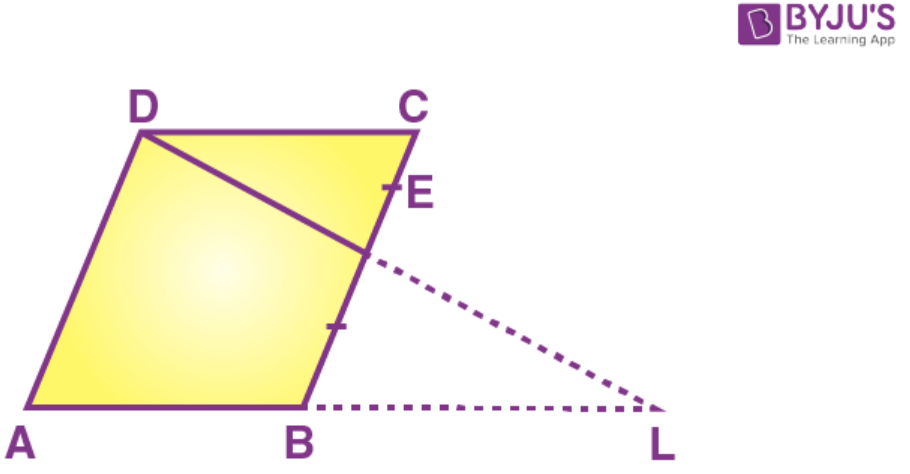Solution:

Given ABCD is a parallelogram in which E is the midpoint of BC

We need to prove that

(i)  ∆ DCE ∆ LBE

(ii) AB = BL.

(iii) AL = 2DC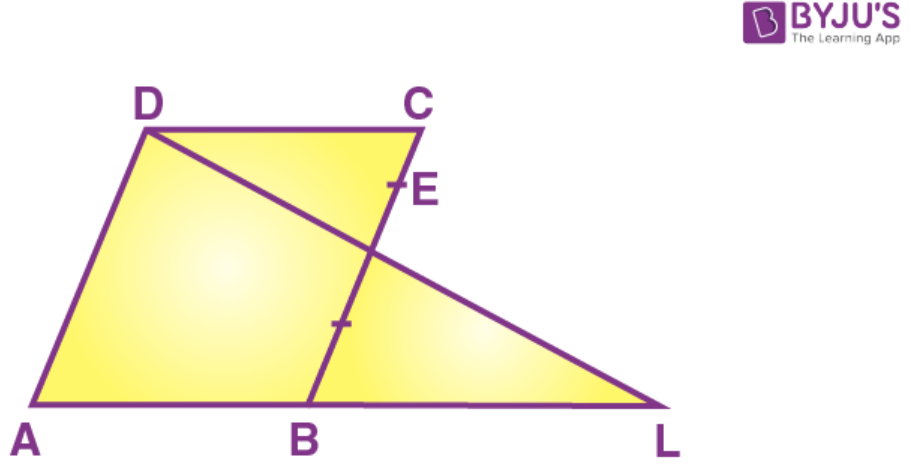(i) In ∆ DCE and ∆ LBE

∠DCE = ∠LBE (DC parallel to AB, alternate angles)

CE = EB (E is the midpoint of BC)

∠DCE = ∠LBE (vertically opposite angles)

By ASA postulate of congruent triangles

∆ DCE ∆ LBE

The corresponding parts of the congruent triangles are congruent

Therefore DC = LB…. (i)

(ii) DC = AB…… (ii)

From (i) and (ii) AB = BL…… (iii)

(iii) AL = AB + BL……… (iv)

From (iii) and (iv) AL = AB + AB

AL = 2AB

AL = 2DC from (ii)

10. In the given figure, AB = DB and Ac = DC.

If ∠ABD = 58o,

∠ DBC = (2x – 4)o,

∠ACB = y + 15o and

∠DCB = 63o; find the values of x and y.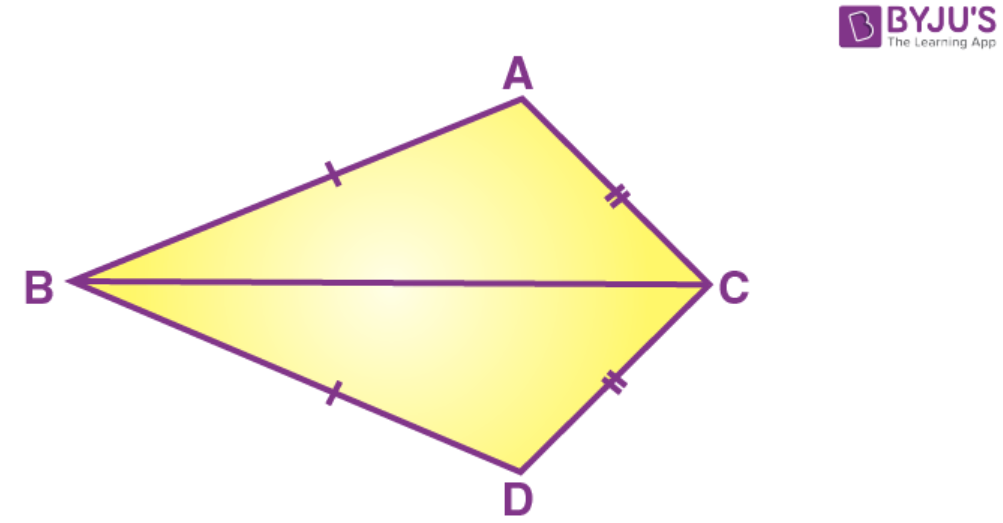Solution:

Given: In the given figure, AB = DB and Ac = DC.

If ∠ABD = 58o,

∠ DBC = (2x – 4)o,

∠ACB = y + 15o and

∠DCB = 63o;

We need to find the values of x and y.

In ∆ ABC and ∆ DBC

AB = DB (given)

AC = DC (given)

BC = BC (common)

By SSS postulate of congruent triangles

∆ ABC ∆ DBC

The corresponding parts of the congruent triangles are congruent

Therefore

∠ACB = ∠DCB

yo + 15o = 63o

yo = 63o – 15o

yo = 48o

∠ACB = ∠DCB (corresponding parts of the congruent triangles)

But ∠DCB = (2x – 4)o

We have ∠ACB + ∠DCB = ∠ABD

(2x – 4)o + (2x – 4)o = 58o

4x – 8o = 58o

4x = 58o + 8o

4x = 66o

x = 66o/4

x = 16.5o

Thus, the values of x and y are

x = 16.5o and y = 48o

Exercise 9B PAGE:125

1. On the sides AB and AC of triangle ABC, equilateral triangle ABD and ACE are drawn.

Prove that:

(ii) CD = BE.

Solution:

Given triangle ABD is an equilateral triangle

Triangle ACE is an equilateral triangle

Now, we need to prove that

(ii) CD = BE.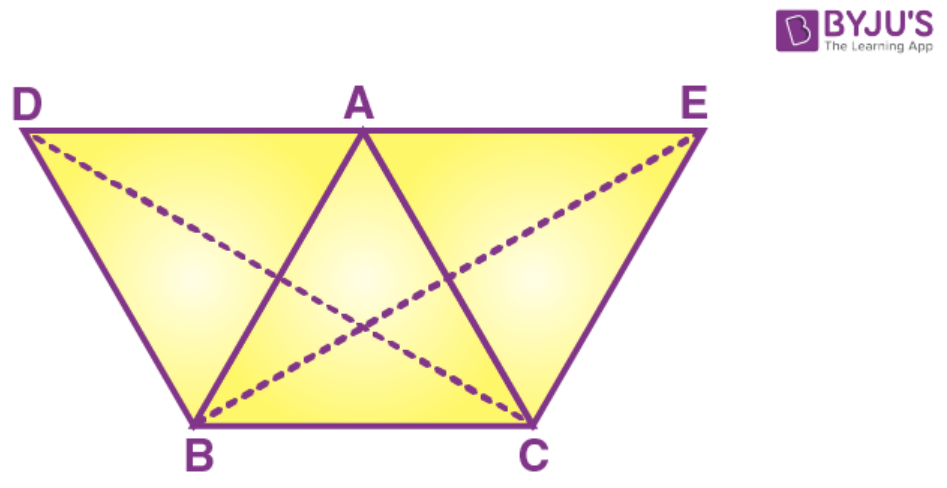Proof:

(i) ∆ ABD is equilateral

Each angle = 60o

Similarly,

∆ ACE is equilateral

Each angle = 60o

∠CAE = 60o ………..(ii)

∠BAD = ∠CAE from (i) and (ii)………..(iii)

Adding ∠BAC to both sides, we have

∠BAD + ∠BAC = ∠CAE + ∠BAC

(ii) In ∆ CAD and ∆ BAE

AC = AE (triangle ACE is equilateral)

AD = AB (triangle ABD is equilateral)

By SAS postulate of congruent triangles

The corresponding parts of the congruent triangles are congruent

Therefore CD = BE

Hence the proof.

2. In the following diagrams, ABCD is a square and APB is an equilateral triangle.

In each case,

(i) Prove that: ∆ APD ≅ ∆ BPC

(ii) Find the angles of ∆DPC.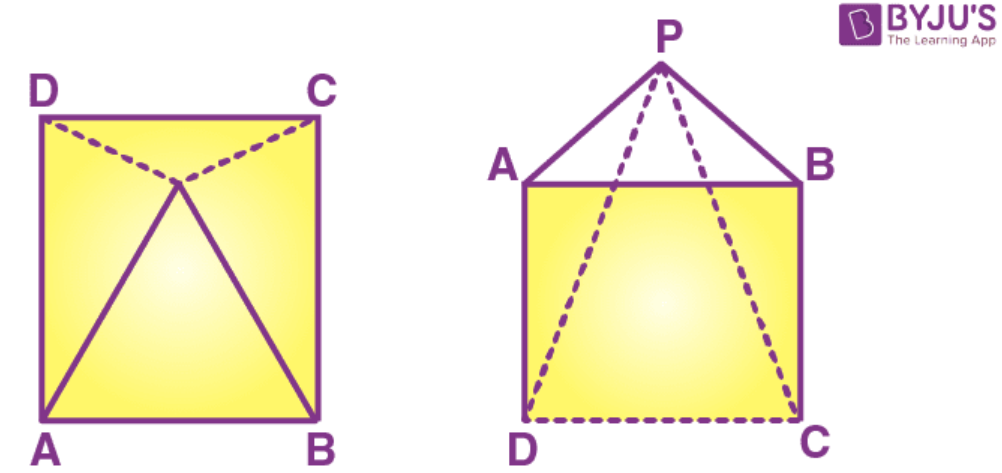Solution:

(a)

(i) Proof:

AP = PB = AB [∆ APB is an equilateral triangle]

Also we have,

∠PBA = ∠PAB = ∠APB = 60o …….. (1)

Since ABCD is a square, we have

∠A = ∠B = ∠C = ∠D = 90o ……….. (2)

Since ∠DAP = ∠A – ∠PAB ………….. (3)

∠DAP = 90o – 60o

∠DAP = 30o [from equation 1 and equation 2] …………… (4)

Similarly ∠CBP = ∠B – ∠PBA

∠CBP = 90o – 60o

∠CBP = 30o [from equation 1 and equation 2] ………… (5)

∠DAP = ∠CBP [from equation 4 and equation 5] ……. (6)

∆ APD and ∆ BPC

AD = BC [sides of square ABCD]

∠DAP = ∠CBP [from 6]

AP = BP [sides of equilateral triangle APB]

Therefore by SAS criteria of congruency, we have

∆ APD ≅ ∆ BPC

(ii) AP = PB = AB [∆ APB is an equilateral triangle] ……… (7)

AB = BC = CD = DA [sides of square ABCD] ………….. (8)

From equation 7 and 8, we have

AP = DA and PB = BC ……… (9)

In ∆ APD,

AP = DA [from 9]

∠ADP = ∠APD [angles opposite to equal sides are equal]

∠ADP + ∠APD + ∠DAP = 180o [sum of angles of a triangle = 180o]

∠ADP + ∠APD + 30o = 180o

∠ADP + ∠ADP = 180o – 30o [from 2 and from 10]

We have ∠PCD = ∠C – ∠PCB

∠PCD = 90o – 75o

∠PCD = 15o ……… (13)

In triangle DPC

∠PDC = 15o

∠PCD = 15o

∠PCD + ∠PDC + ∠DPC = 180o

∠DPC = 180o – 30o

∠DPC = 150o

Therefore angles are 15o, 150o and 15o

(b)

(i) Proof: In triangle APB

AP = PB = AB

Also,

We have,

∠PBA = ∠PAB = ∠APB = 60o ……… (1)

Since ABCD is a square, we have

∠A = ∠B = ∠C = ∠D = 90o ………. (2)

∠DAP = ∠A + ∠PAB…….. (3)

∠DAP = 90o + 60o

∠DAP = 150o [from 1 and 2] ……. (4)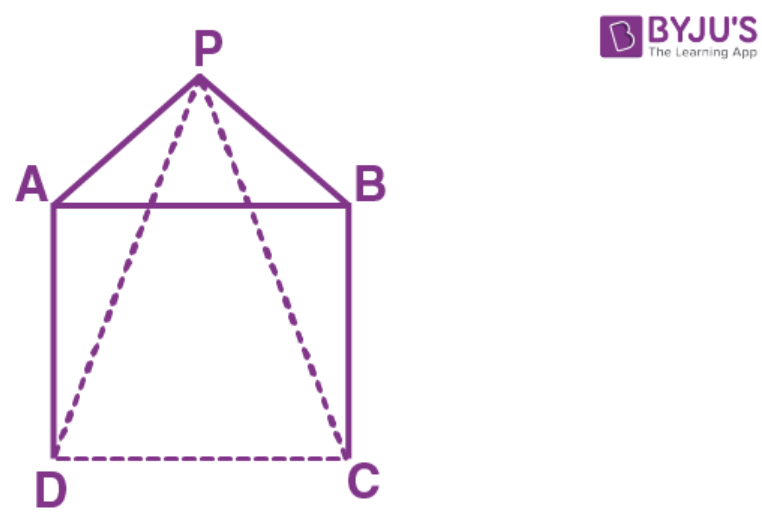∠CBP = ∠B + ∠PBA…….. (3)

∠CBP = 90o + 60o

∠CBP = 150o [from 1 and 2] …… (5)

∠DAP = ∠CBP [from 4 and 5] …….. (6)

In triangle APD and triangle BPC

AD = BC [sides of square ABCD]

∠DAP = ∠CBP [from 6]

AP = BP [sides of equilateral triangle APB]

By SAS criteria we have

∆ APD ≅ ∆ BPC

(ii) AP = PB = AB [triangle APB is an equilateral triangle] ……… (7)

AB = BC = CD = DA [sides of square ABCD] ………….. (8)

From equation 7 and 8, we have

AP = DA and PB = BC ……… (9)

In ∆ APD,

AP = DA [from 9]

∠ADP = ∠APD [angles opposite to equal sides are equal] ……….. (10)

∠ADP + ∠APD + ∠DAP = 180o [sum of angles of a triangle = 180o]

∠ADP + ∠APD + 150o = 180o

∠ADP + ∠ADP = 180o – 150o [from 2 and from 10]

We have ∠PCD = ∠D – ∠ADP

∠PCD = 90o – 15o

∠PCD = 75o ……… (11)

In triangle BPC

PB = BC [from 9]

∠PCB = ∠BPC ……… (12)

∠PCB + ∠BPC + ∠CPB = 180o

∠PCB + ∠PCB = 180o – 150o [from 2 and from 10]

2 ∠PCB = 30o

∠PCB = 15o

We have ∠PCD = ∠C – ∠PCB

∠PCD = 90o – 15o

∠PCD = 75o ……… (11)

In triangle DPC

∠PDC = 75o

∠PCD = 75o

∠PCD + ∠PDC + ∠DPC = 180o

75o + 75o + ∠DPC = 180o

∠DPC = 180o – 150o

∠DPC = 30o

Angles of triangle are 75o, 30o and 75o

3. In the figure, given below, triangle ABC is right-angled at B. ABPQ and ACRS are squares. Prove that:

(i) ∆ACQ and ∆ASB are congruent.

(ii) CQ = BS.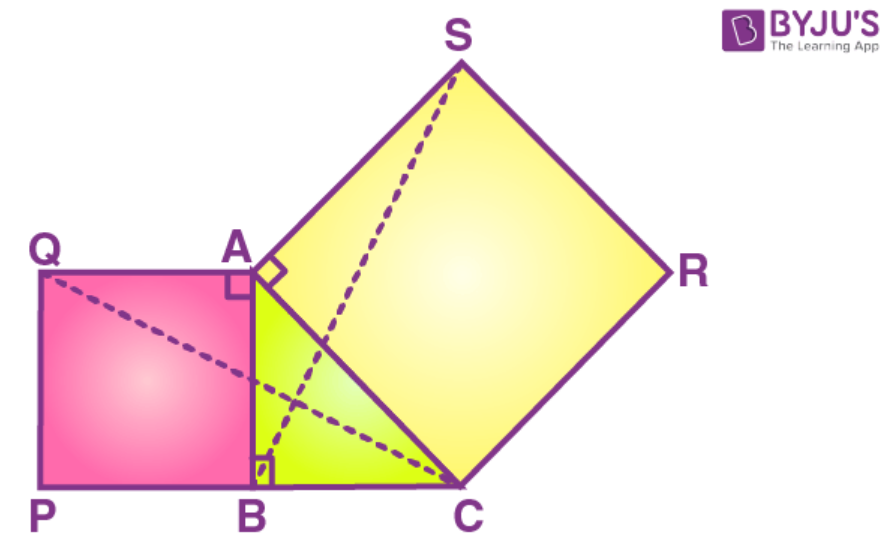Solution:

Triangle ABC is right-angled at B.

ABPQ and ACRS are squares.

We need to prove that:

(i) ∆ACQ and ∆ASB are congruent.

(ii) CQ = BS.

Proof:

(i) ∠QAB = 90o (ABPQ is a square) …. (1)

∠SAC = 90o (ACRS is a square) ………. (2)

From (1) and (2) we have

∠QAB = ∠SAC ………… (3)

Adding ∠BAC both sides of (3) we get

∠QAB + ∠BAC = ∠SAC + ∠BAC

∠QAC = ∠SAB ………. (4)

In ∆ ACQ and ∆ ASB

QA = QB (sides of a square ABPQ)

AC = AS (side of a square ACRS)

By AAS postulate of congruent triangles

Therefore ∆ ACQ ∆ ASB

(ii)The corresponding parts of the congruent triangles are congruent

Therefore CQ = BS

4. In a ∆ABC, BD is the median to the side AC, BD is produced to E such that BD = DE. Prove that: AE is parallel to BC.

Solution:

Given in a ∆ABC, BD is the median to the side AC,

BD is produced to E such that BD = DE.

Now we have to prove that: AE is parallel to BC.

Construction: Join AE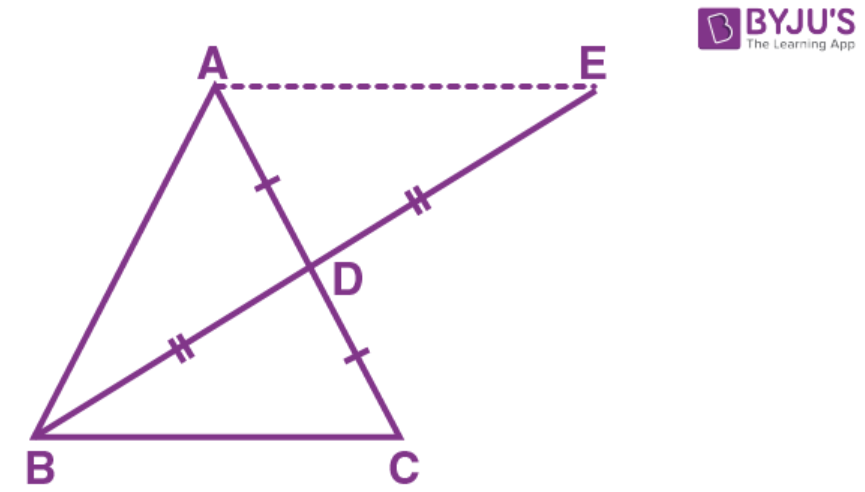Proof:

AD = DC (BD is median to AC)

In ∆ BDC and ∆ ADE

BD = DE (Given)

∠BDC = ∠ADE = 90o (vertically opposite angles)

By SAS postulate of congruent triangles

The corresponding parts of the congruent triangles are congruent

But these are alternate angles

And AC is the transversal

Thus, AE parallel to BC

5. In the adjoining figure, OX and RX are the bisectors of the angles Q and R respectively of the triangle PQR.

If XS ⊥ QR and XT ⊥ PQ; prove that:

(i) ∆ XTQ ≅ ∆ XSQ

(ii) PX bisects angle ∠P.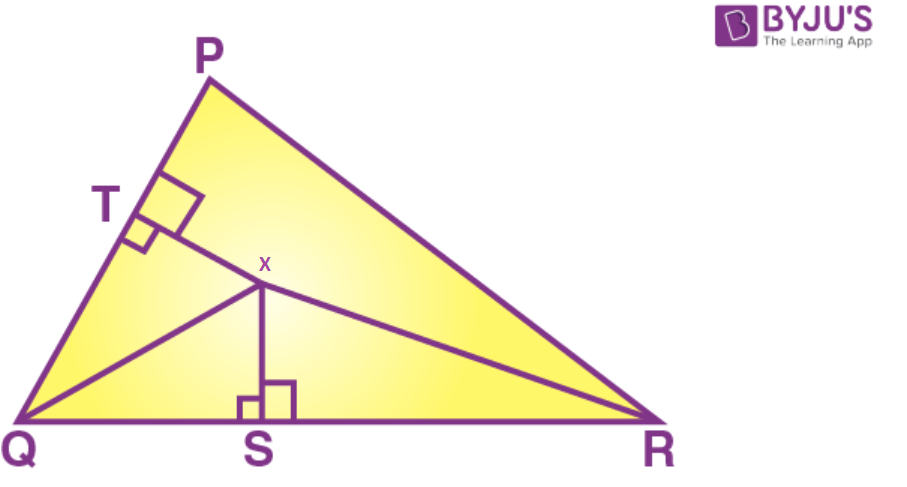Solution:

OX and RX are the bisectors of the angles Q and R respectively of the triangle PQR.

If XS  QR and XT  PQ;

We have to prove that:

(i) ∆ XTQ ∆ XSQ

(ii) PX bisects angle ∠P.

Construction:

Draw If XZ PR and join PX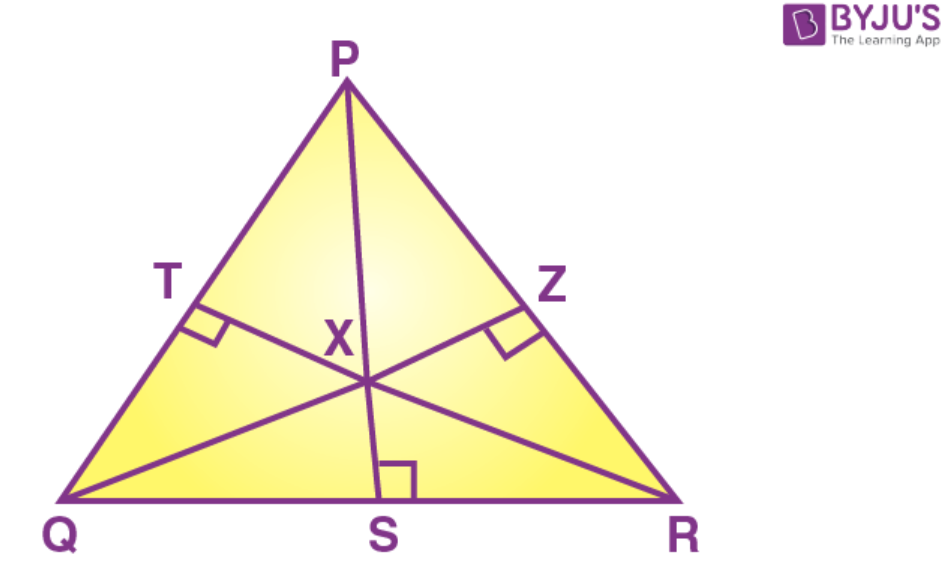Proof:

(i) In ∆ XTQ and ∆ XSQ

∠QTX = ∠QSX = 90o (XS perpendicular to QR and XT perpendicular to PQ)

∠QTX = ∠QSX (QX is bisector of angle Q)

QX = QX (common)

By AAS postulate of congruent triangles

Therefore ∆ XTQ ∆ XSQ ………….. (1)

(ii) The corresponding parts of the congruent triangles are congruent

Therefore XT = XS (by c.p.c.t)

In ∆ XSR and ∆ XZR

∠XSR = ∠XZR = 90o (XS perpendicular to XS and angle XSR = 90o)

∠SRX = ∠ZRX (RX is a bisector of angle R)

RX = RX (common)

By AAS postulate of congruent triangles

Therefore ∆ XSR ∆ XZR ………….. (1)

The corresponding parts of the congruent triangles are congruent

Therefore XS = XZ (by c.p.c.t) ……….. (2)

From (1) and (2)

XT = XZ …………… (3)

In ∆ XTP and ∆ XZP

∠XTP = ∠XZP = 90o (Given)

XP = XP (common)

XT = XZ (from 3)

By right angle hypotenuse side postulate of congruent triangles

Therefore ∆ XTP ∆ XZP

The corresponding parts of the congruent triangles are congruent

∠XPT = ∠XPZ

PX bisects ∠SRX = ∠P

6. In the parallelogram ABCD, the angles A and C are obtuse. Points X and Y are taken on the diagonal BD such that the angles XAD and YCB are right angles.

Prove that: XA = YC.

Solution:

ABCD is a parallelogram in which ∠A and ∠C are obtuse.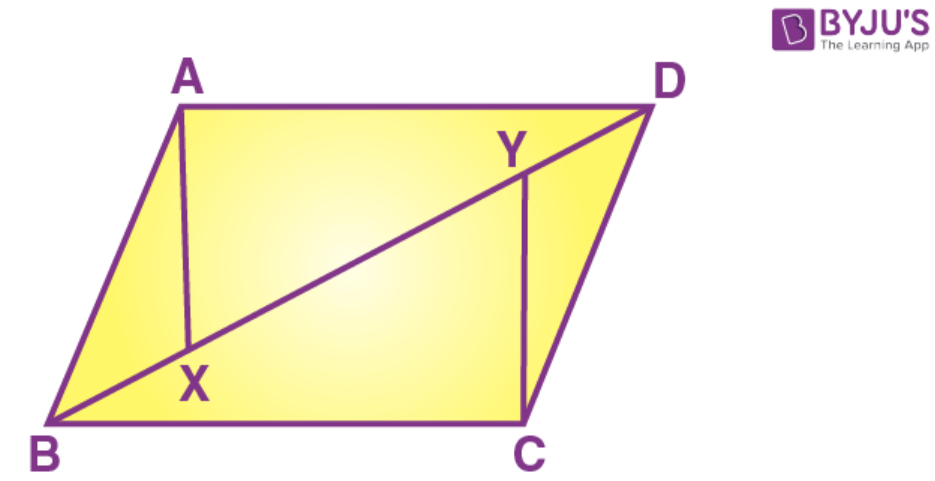Points X and Y are on the diagonal BD

Such that ∠XAD = ∠YCB = 90o

We need to prove that XA = YC

Proof:

In ∆ XAD and ∆ YCB

∠XAD = ∠YCB = 90o (Given)

AD = BC (opposite sides of a parallelogram)

By ASA postulate of congruent triangles

The corresponding parts of the congruent triangles are congruent

Therefore XA = YC

Hence the proof.

7. ABCD is a parallelogram. The sides AB and AD are produced to E and F respectively, such produced to E and F respectively, such that AB = BE and AD = DF.

Prove that: ∆ BEC ≅ ∆ DCF

Solution:

ABCD is a parallelogram.

The sides AB and AD are produced to E and F respectively,

Such that AB = BE and AD = DF.

We need to prove that ∆ BEC ∆ DCF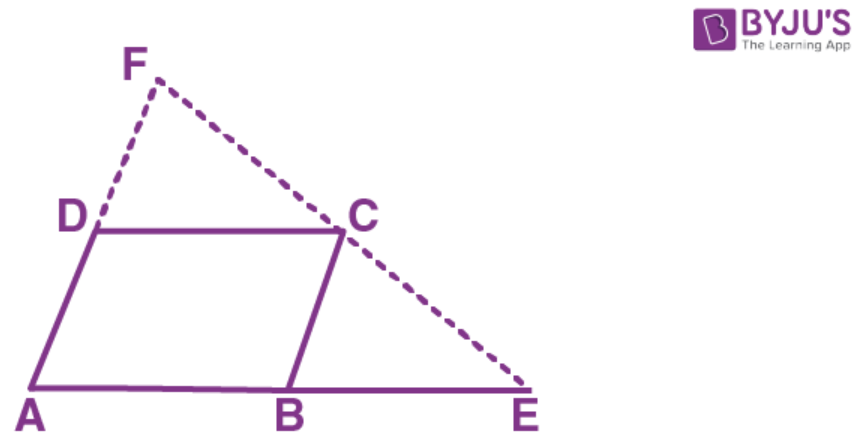Proof:

AB = DC (opposite sides of a parallelogram) ……. (1)

AB = BE (given) ……….. (2)

From (1) and (2) we have

BE = DC (opposite sides of a parallelogram) ………. (3)

AD = BC (opposite sides of a parallelogram) …….. (4)

AD = DF (given) ………… (5)

From (4) and (5) we have

BC = DF ……… (6)

Since AD parallel to BC the corresponding angles are equal

∠DAB = ∠CBE …….. (7)

Since AD parallel to DC the corresponding angles are equal

∠DAB = ∠FDC ……… (8)

From (7) and (8)

∠CBE = ∠FDC …….. (9)

In ∆ BEC and ∆ DCF

BE = DC (from (3))

∠CBE = ∠FDC (from (9))

BC = DF (from (6))

By SAS postulate of congruent triangles

Therefore ∆ BEC ∆ DCF

Hence the proof.

8. In the following figures, the sides AB and BC and the median AD of triangle ABC are equal to the sides PQ and QR and median PS of the triangle PQR. Prove that ∆ABC and ∆PQR are congruent.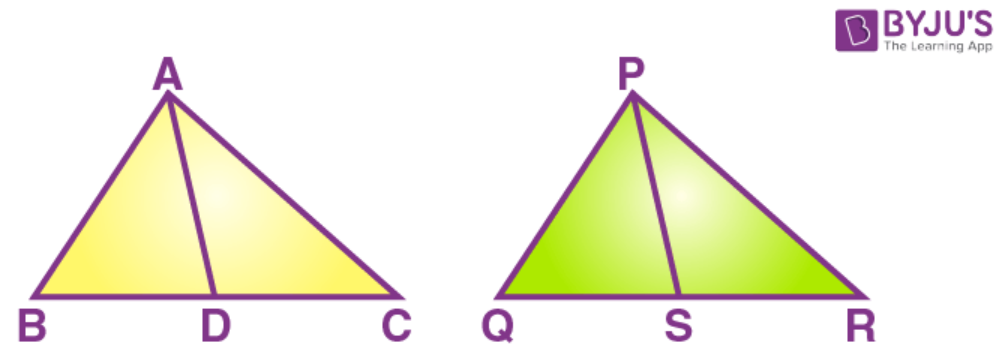Solution:

Since BC = QR

We have BD = QS and DC = SR (D is the midpoint of BC and S is the midpoint of QR)

In ∆ ABD and ∆ PQS

AB = PQ ……… (1)

BD = QS ……….. (3)

By SSS postulate of congruent triangles

Therefore ∆ ABD ∆ PQS

Similarly

In ∆ ADC and ∆ PSR

AC = PR ……….. (5)

DC = SR ………. (6)

By SSS postulate of congruent triangles

We have

BC = BD + DC (D is the midpoint of BC)

= QS + SR (from (3) and (6))

= QR (S is the midpoint of QR) ……… (7)

Now again consider the triangles ∆ ABC and ∆ PR

AB = PQ (from 1)

BC = QR (from 7)

AC = PR (from 7)

By SSS postulate of congruent triangles

Therefore ∆ ABC ∆ PQR

Hence the proof.

9. In the following diagram, AP and BQ are equal and parallel to each other.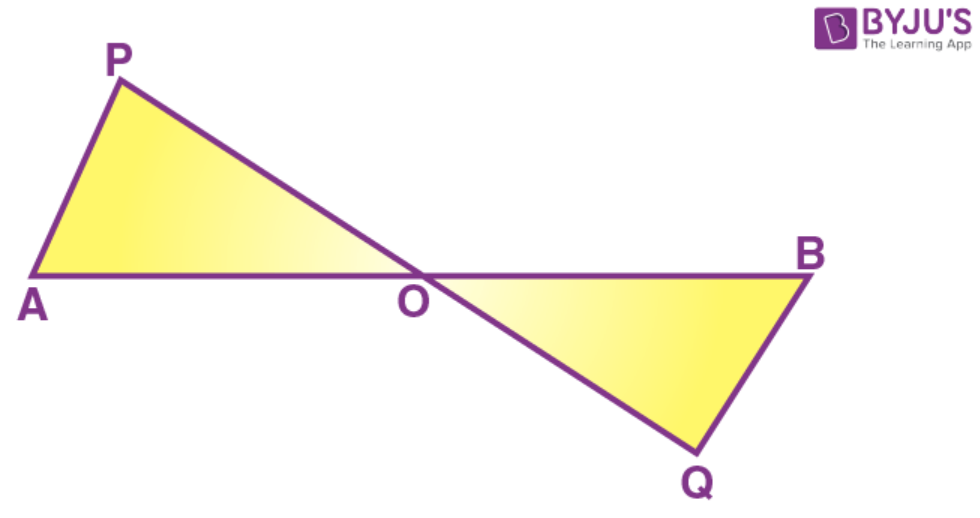Prove that

(i) ∆ AOP ≅ ∆ BOQ

(ii) AB and PQ bisect each other

Solution:

In the figure AP and BQ are equal and parallel to each other

Therefore AP = BQ and AP parallel to BQ

We need to prove that

(i) ∆ AOP ∆ BOQ

(ii) AB and PQ bisect each other

(i) since AP parallel to BQ

∠APO = ∠BQO (alternate angles) …….. (1)

And ∠PAO = ∠QBO (alternate angles) …….. (2)

Now in ∆ AOP and ∆ BOQ

∠APO = ∠BQO (from 1)

AP = PQ (given)

∠PAO = ∠QBO (from 2)

By ASA postulate of congruent triangles,

∆ AOP ∆ BOQ

(ii) the corresponding parts of the congruent triangles are congruent

Therefore OP = OQ (by c.p.c.t)

OA = OB (by c.p.c.t)

Hence AB and PQ bisect each other

10. In the following figure, OA = OC and AB = BC.

(i) ∠P= 90o

(ii) ∆ AOD ≅ ∆ COD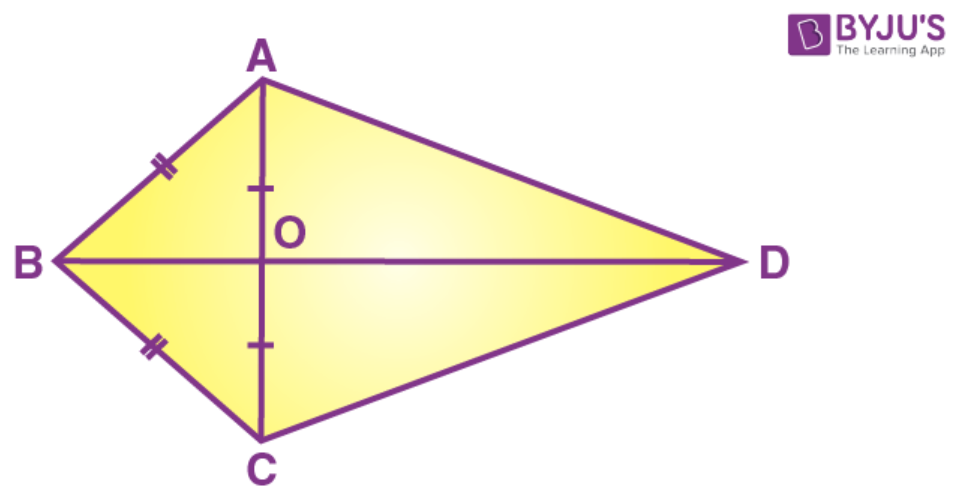Solution:

Given OA = OC and AB = BC.

Now we have to prove that,

(i) ∠P= 90o

(ii) ∆ AOD ∆ COD

(i) In ∆ ABO and ∆ CBO

AB = BC (given)

AO = CO (given)

OB = OB (common)

By SSS postulate of congruent triangles

Therefore ∆ ABO ∆ CBO

The corresponding parts of the congruent triangles are congruent

∠ABO = ∠CBO (by c.p.c.t)Hence∠ABD = ∠CBD

∠AOB = ∠CBO (by c.p.c.t)

We have ∠ABO + ∠CBO = 180o (linear pair)

∠ABO = ∠CBO = 90o

And AC perpendicular to BD

(ii) In ∆ AOD and ∆ COD

OD = OD (common)

∠AOD = ∠COD (each = 90o)

AO = CO (given)

By SAS postulate of congruent triangles

Therefore ∆ AOD ∆ COD

(iii) The corresponding parts of the congruent triangles are congruent

Therefore AD = CD (by c.p.c.t)

Hence the proof.

## Selina Solutions for Class 9 Maths chapter 9- Triangles

The chapter 9, Triangles, contains 2 exercises and the Solutions given here contains the answers for all the questions present in these exercises. Let us have a look at some of the topics that are being discussed in this chapter.

9.1 Introduction

9.2 Relation between sides and angles of triangles

9.3 Some important terms

9.4 Congruent Triangles

9.5 Conditions for congruency of triangles

## Selina Solutions for Class 9 Maths chapter 9- Triangles

The chapter 9 of class 9 deals about triangles. A triangle is a plane figure bounded by three straight line segments. Every triangle has three vertices and three sides.the chapter also explains about different terms like median, centroid, altitud, orthocentre etc. Read and learn the chapter 9 of Selina textbook to get to know more about Triangles. Learn the Selina Solutions for Class 9 effectively to attain excellent result in the examination.